## Sunday, December 30, 2018

2015
(November)
MATHEMATICS
(Major)
Course: 501
(Logic and Combinatorics, and Analysis - III)
Full Marks: 80
Pass Marks: 32
Time: 3 hours
The figures in the margin indicate full marks for the questions
(A) Logic and Combinatorics
(Marks: 35)
1. (a) Define a truth function. 1
(b) Let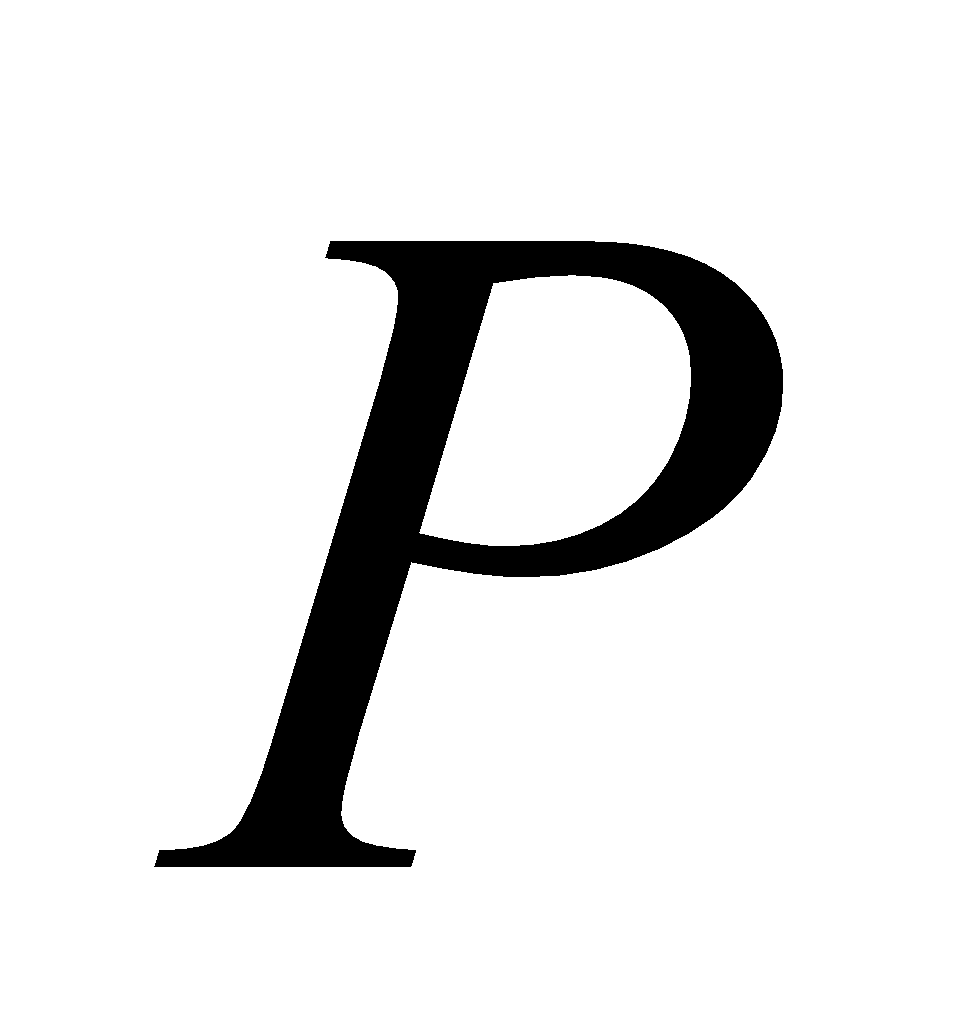: Ice is cold,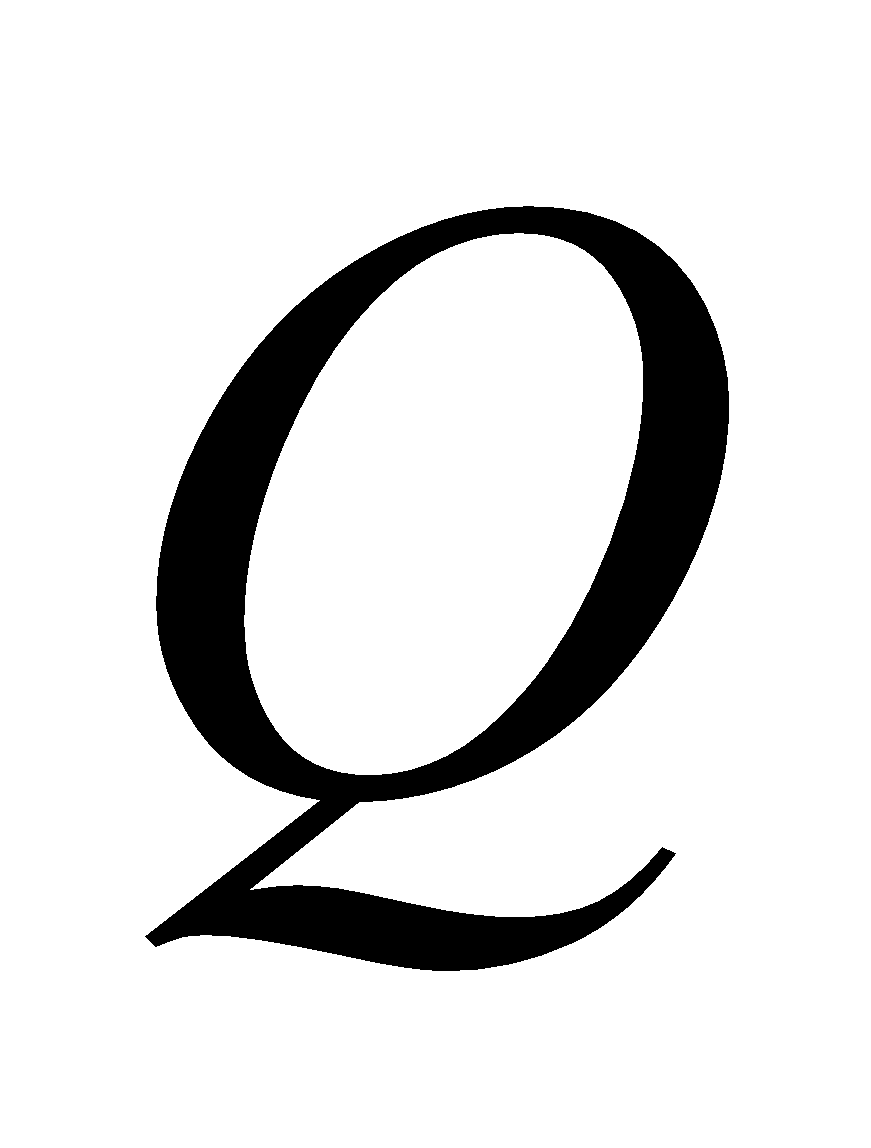: Blood is green. Write the following sentences in symbolic form: 2
1. Either ice is cold or blood is green.
2. If blood is green then ice is not cold.
(c) Prove that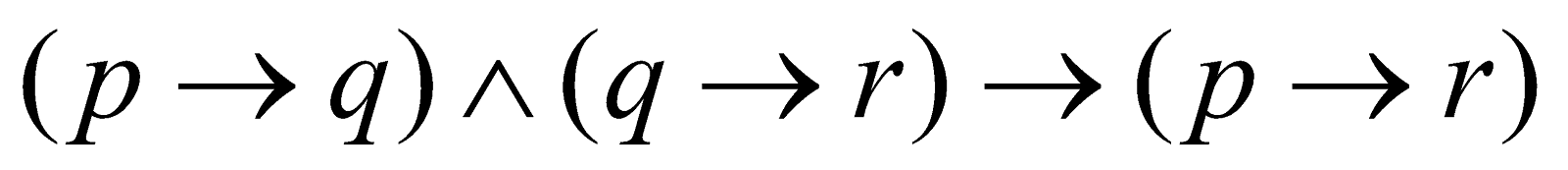is a tautology. 3
(d) Prove that every statement can be generated by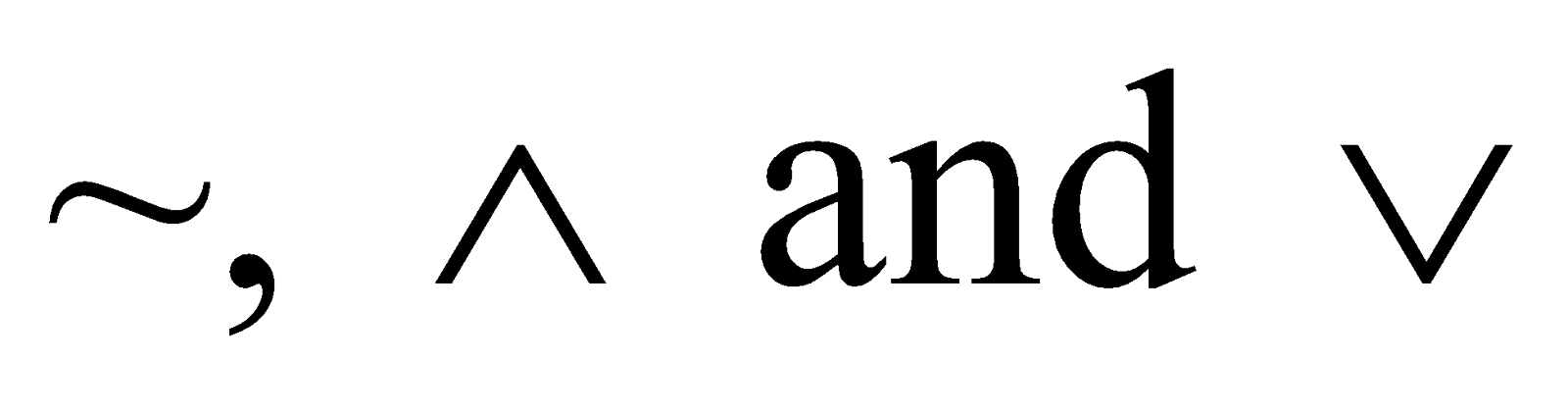only. 4
Or
Prove that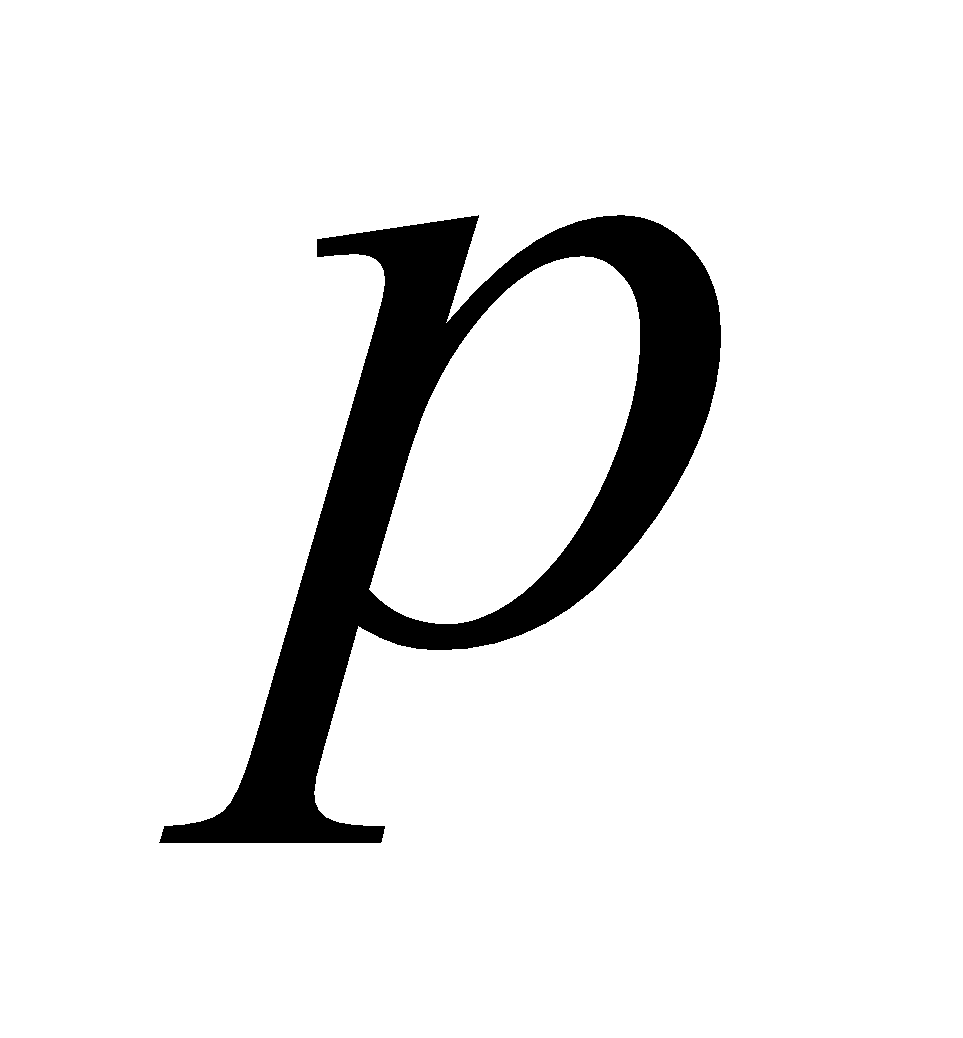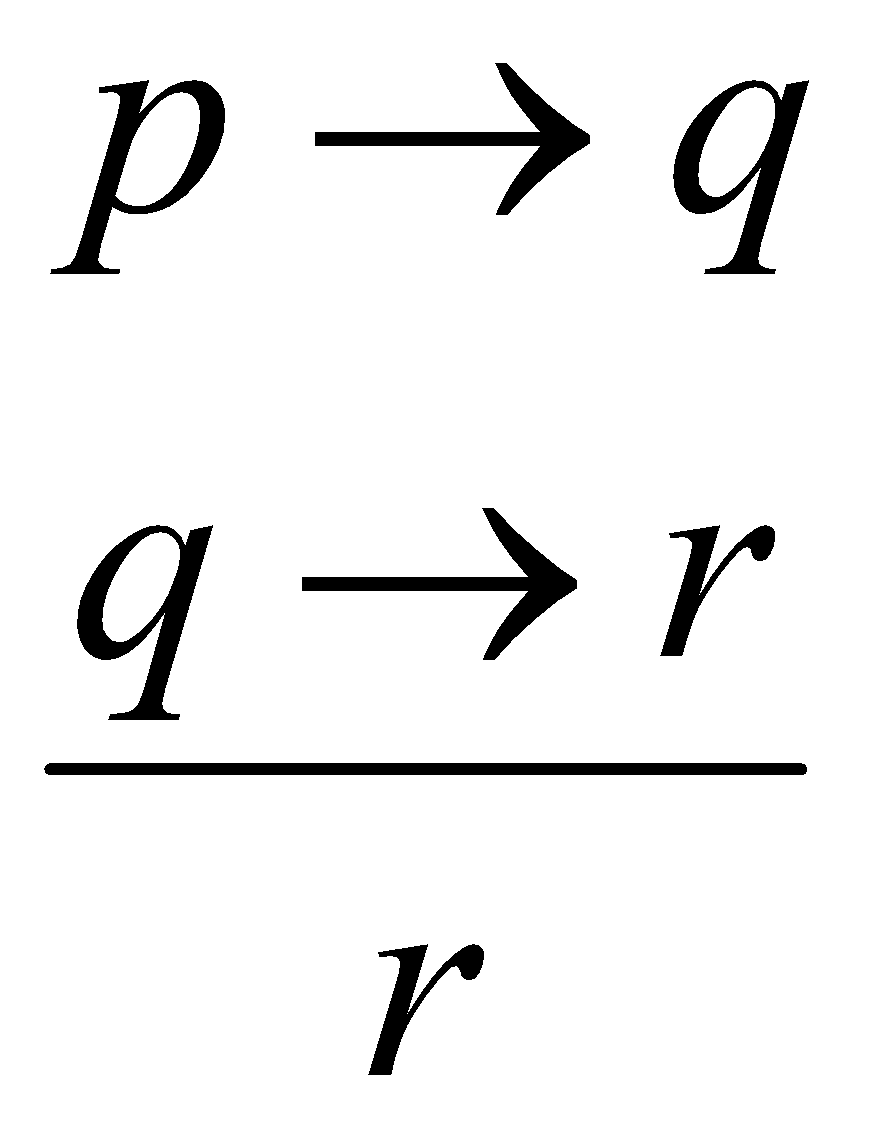is a valid statement. 4
2. (a) Write the law of syllogism. 1
(b) Using predicates, write the following sentences in symbolic form: 2
1. All teachers like all students.
2. Only teachers like students.
(c) Test the validity of the following arguments: 3
All squares have equal sides.
A rhombus has equal sides.
Therefore, a rhombus is a square.
(d) Prove that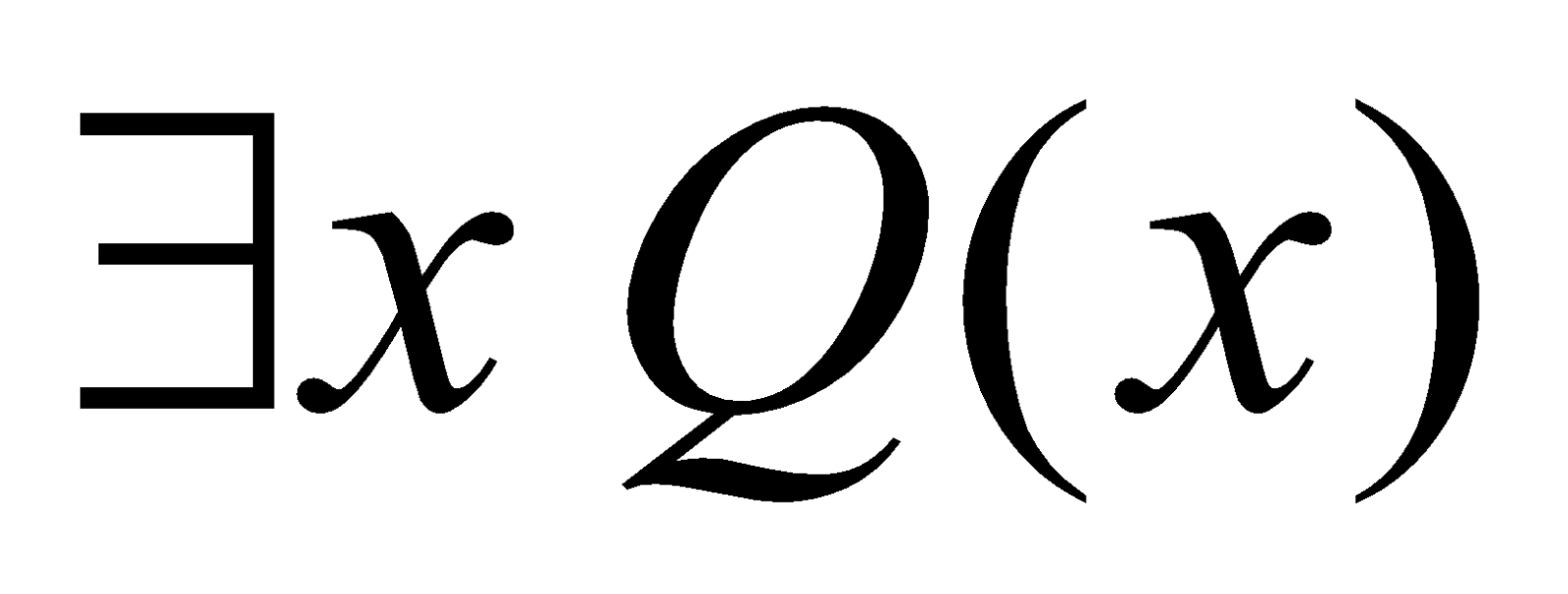is a valid consequence of the following premises: 4
1.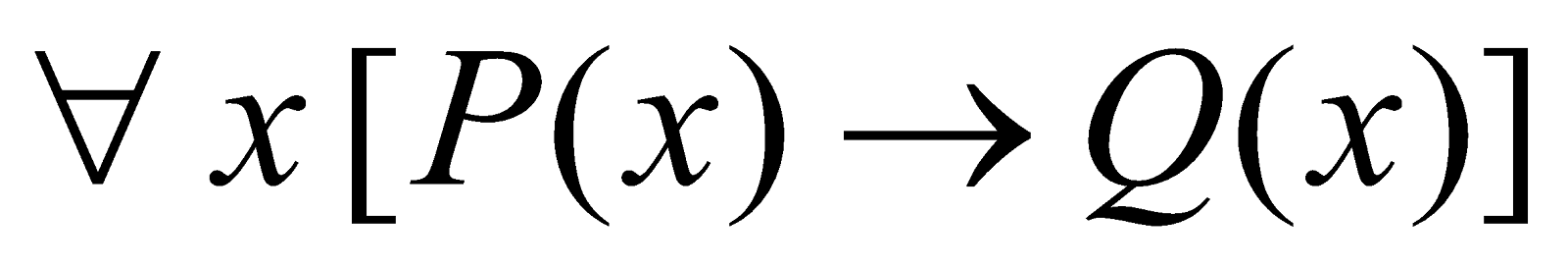2.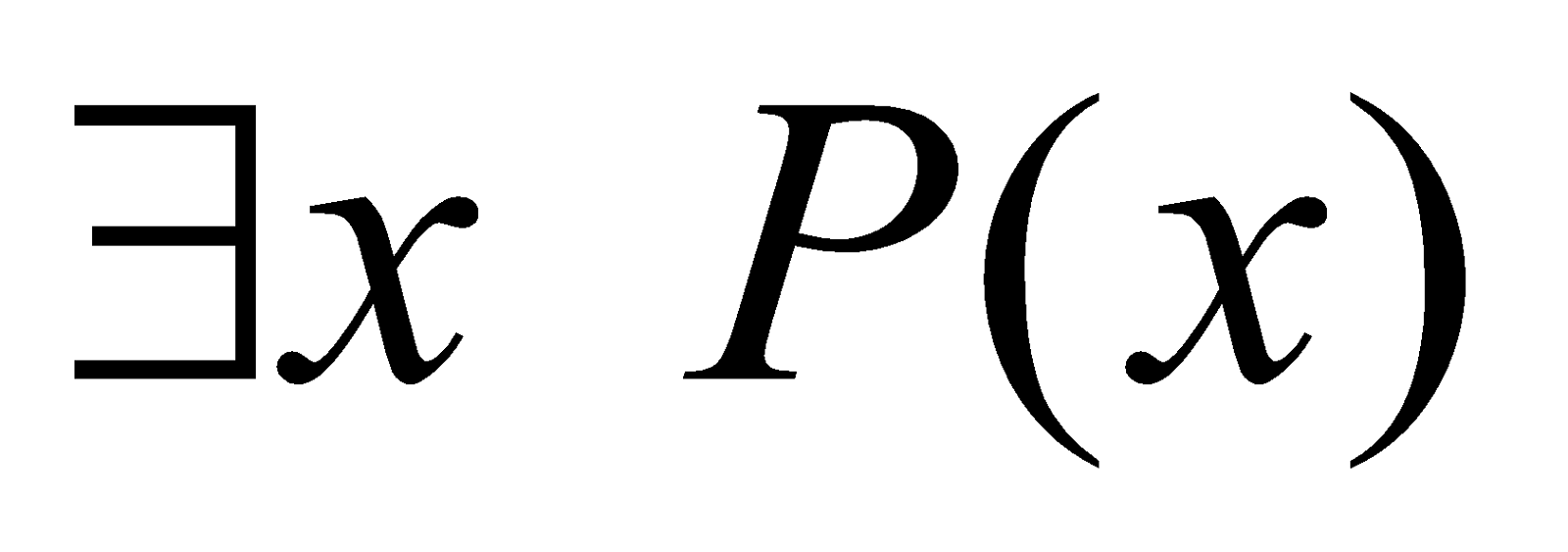Or
All men are mortal.
Ram is a man.
Hence Ram is mortal.
Write the formal derivation.
3. (a) Write the fundamental principles of counting. 1
(b) A computer password consists of a letter of the alphabet followed by 3 or 4 digits. Find the total number of passwords that can be formed. 2
(c) Define Catalan number. Prove that the nth Catalan number defined fromto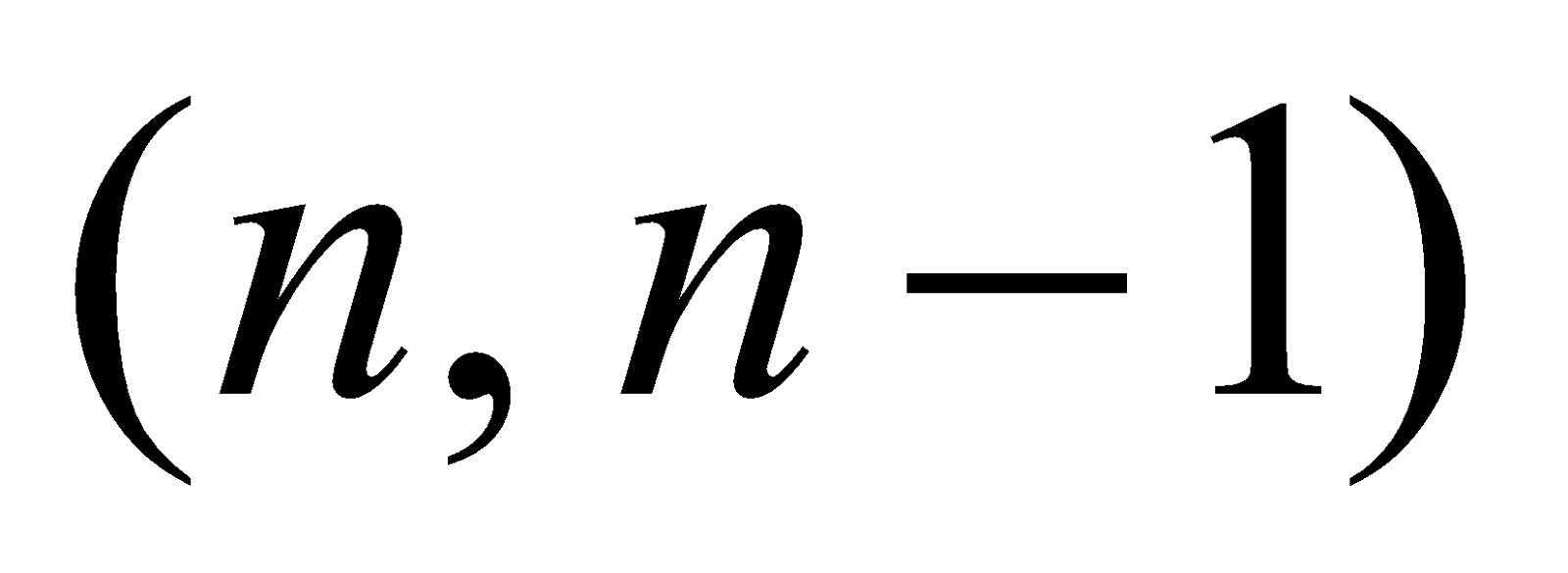is given by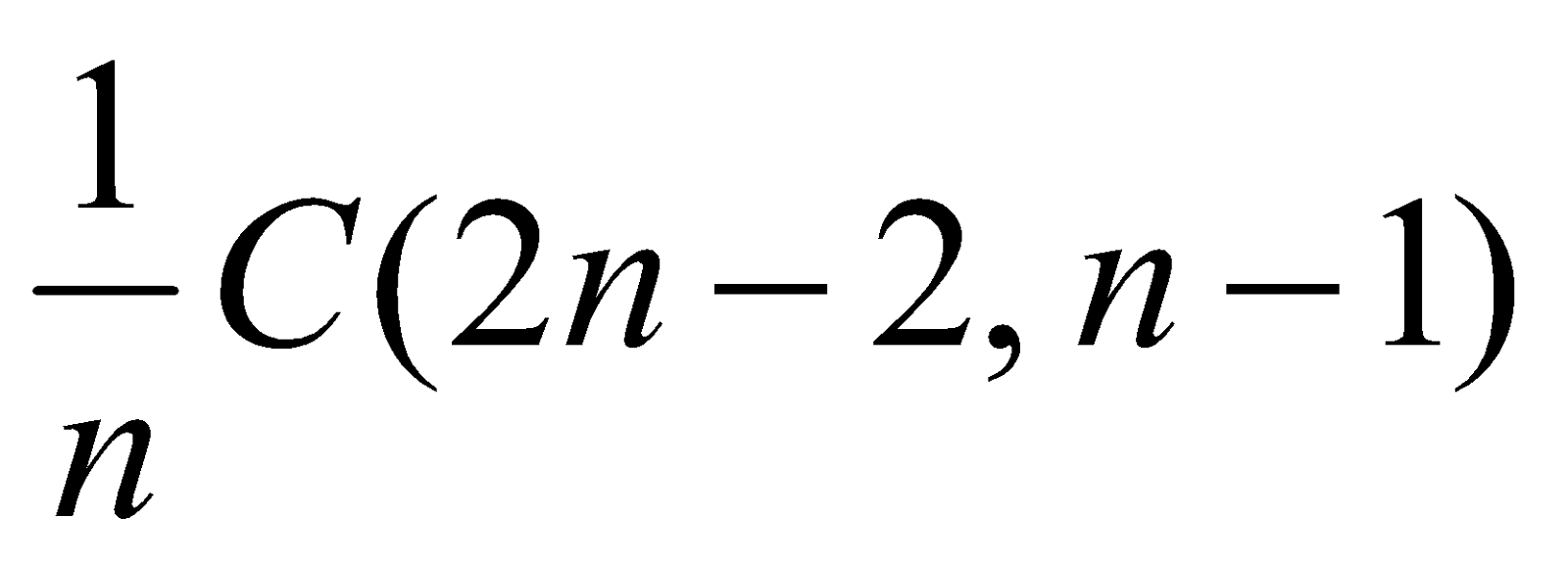1+3=4
Or
Define Stirling number of first kind. Find the number of functions from a set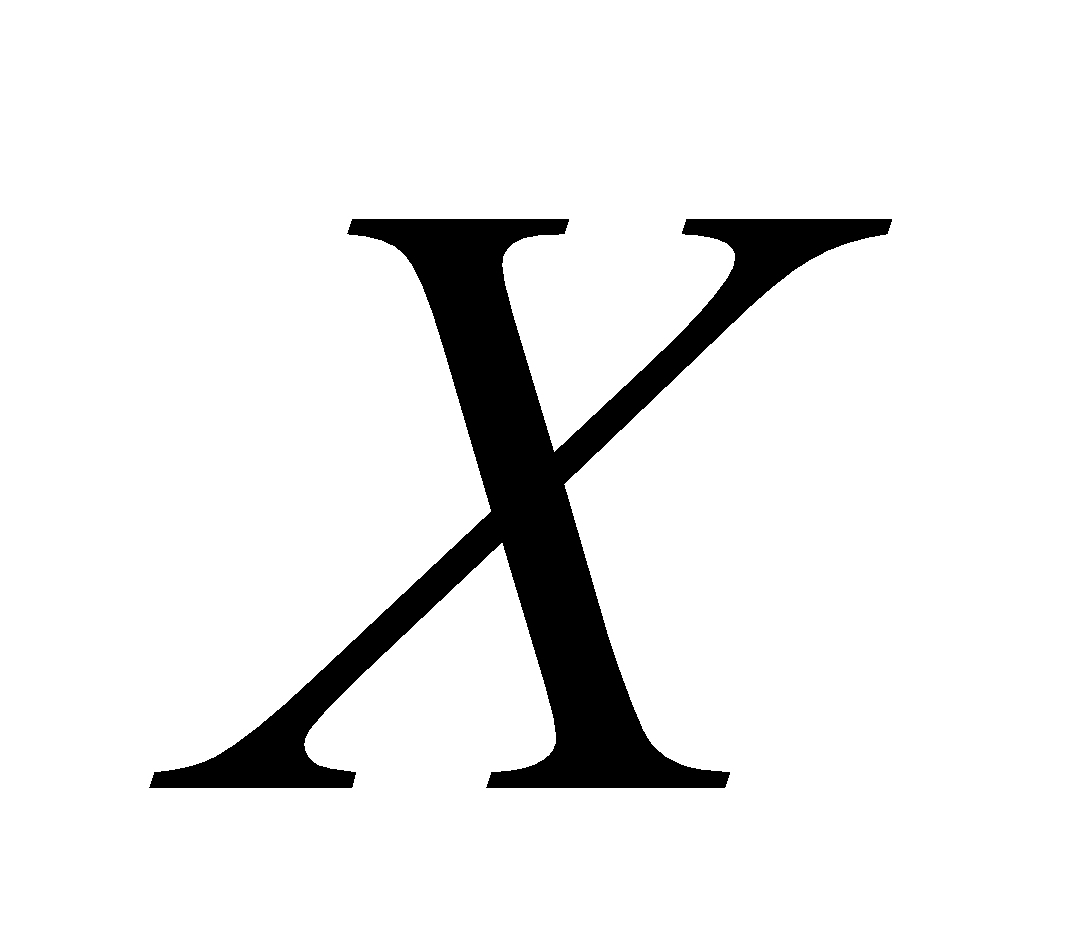of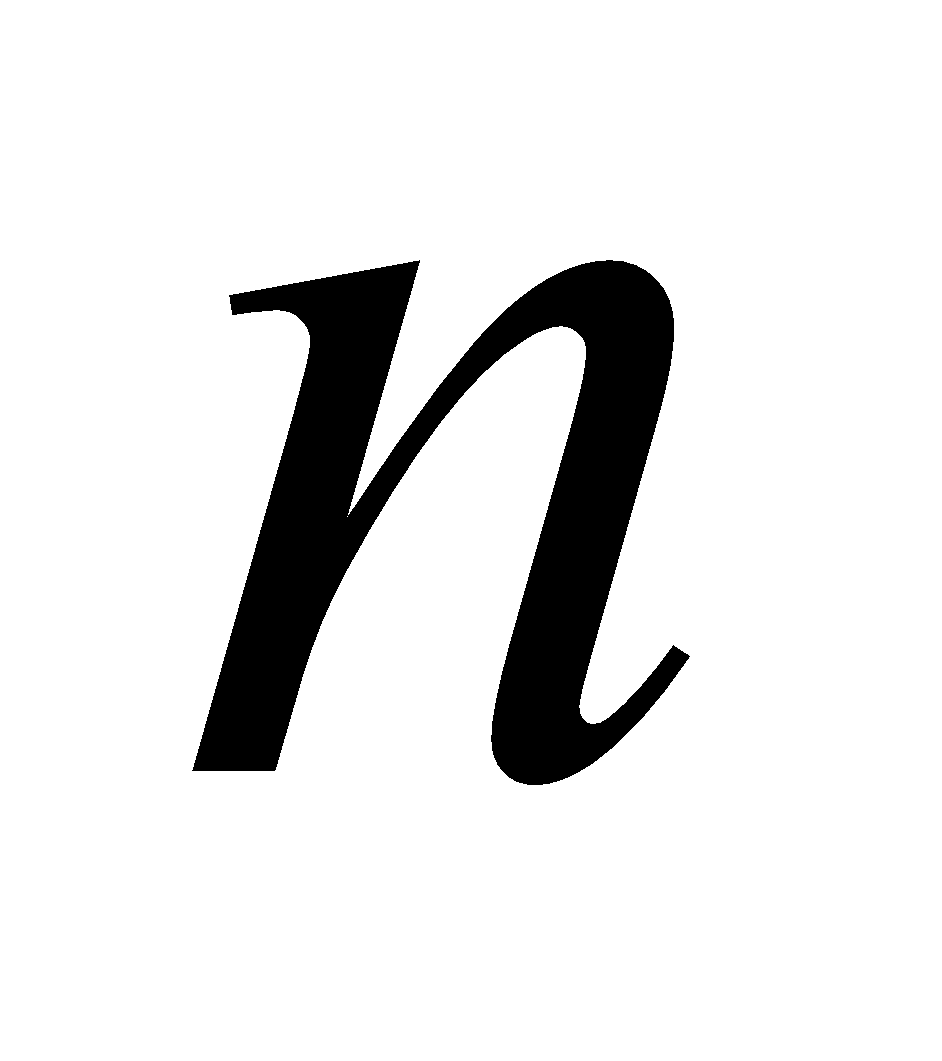elements to a set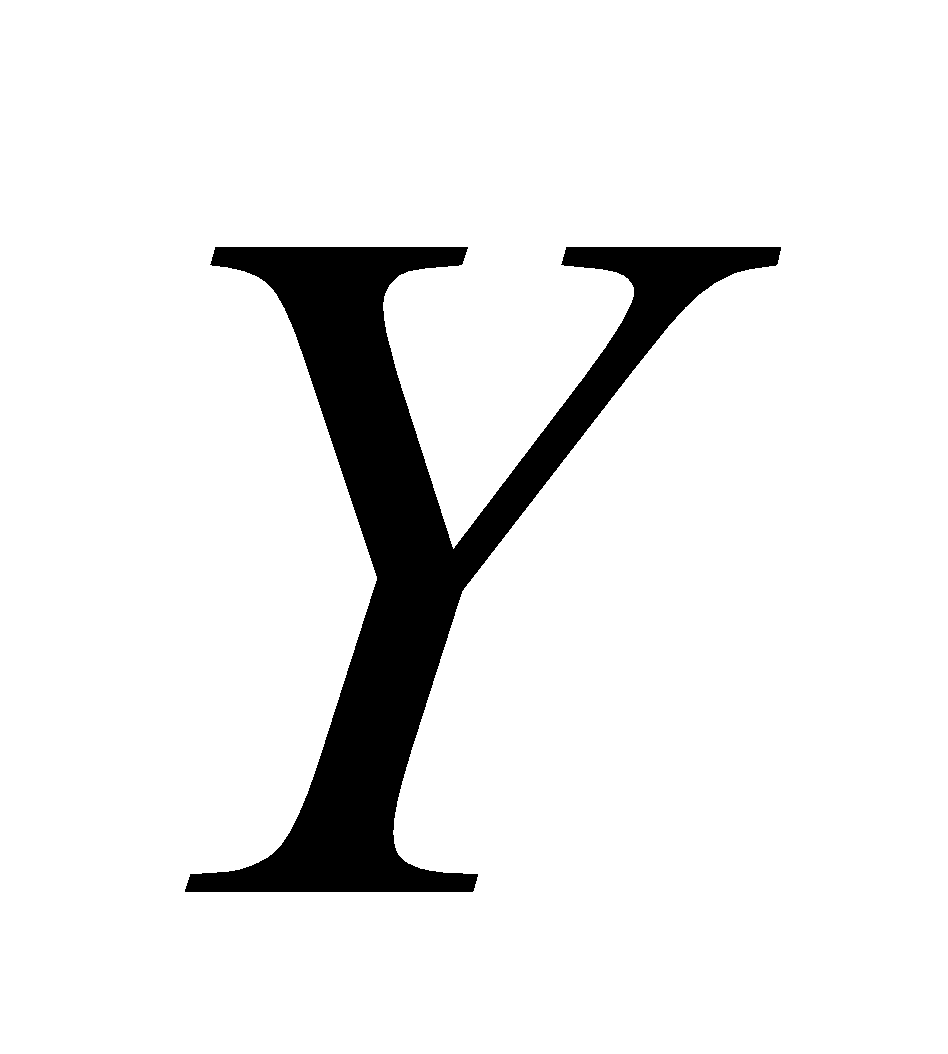of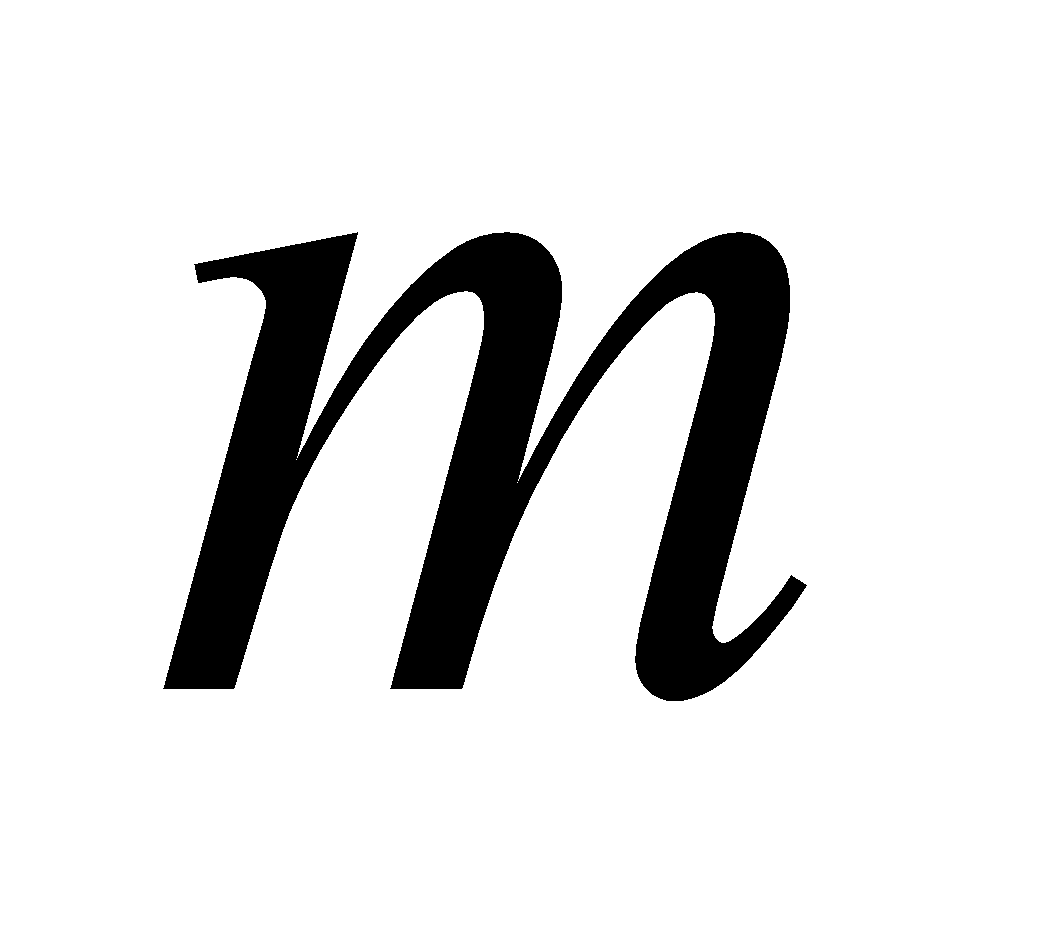elements such that the ranges of these functions have exactly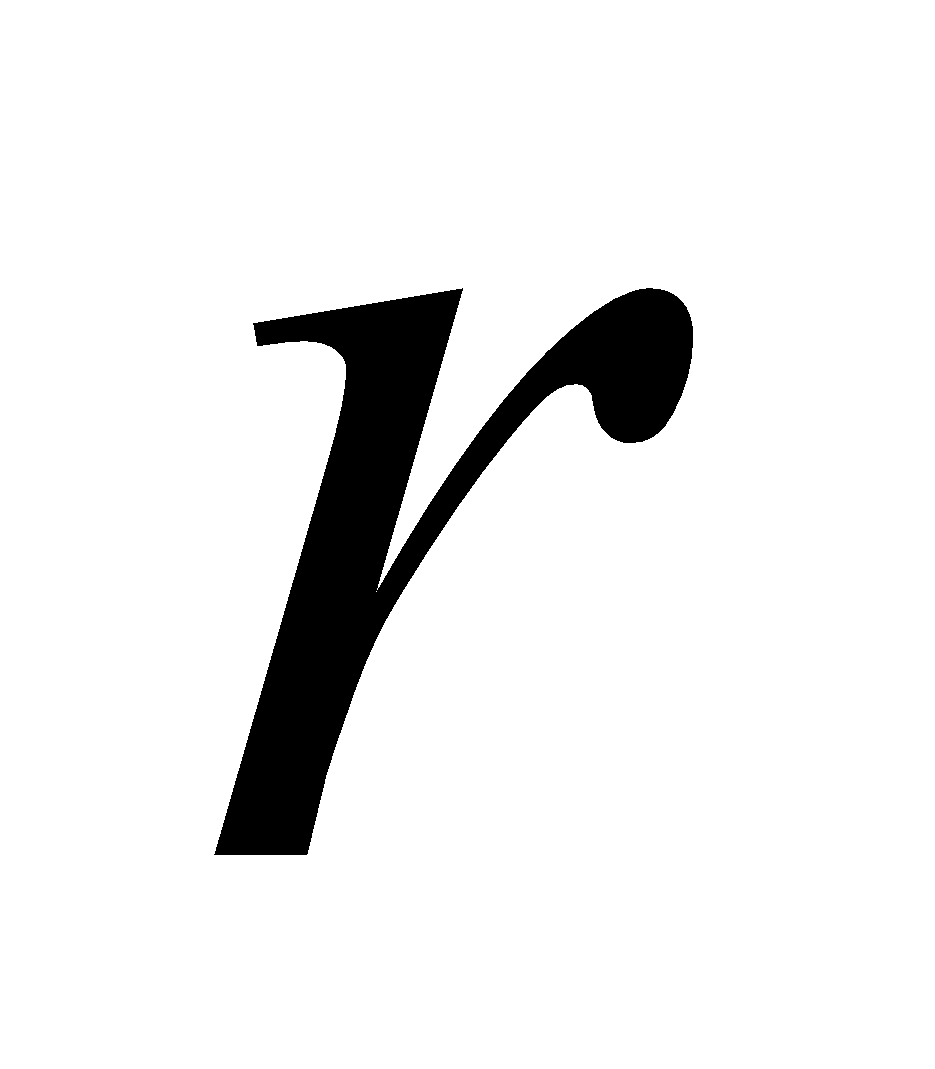elements each. 4
4. (a) Write the Pigeonhole theorem. 1
(b) Prove that given any 12 natural numbers, one can choose 2 of them such that their difference is divisible by 11.     3
(c) Find the number of solutions in integers of the equation 4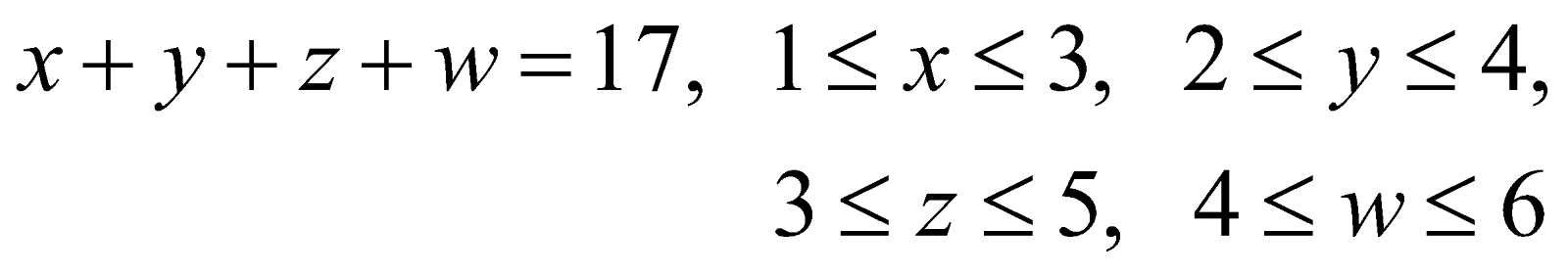Or
Use generating functions to find the number of ways to selectobjects ofdifferent kinds if we must select at least one object of each kind. 4
(B) Analysis – III (Complex Analysis)
(Marks: 45)
5. (a) Define continuity of a function of a complex variable. 1
(b) If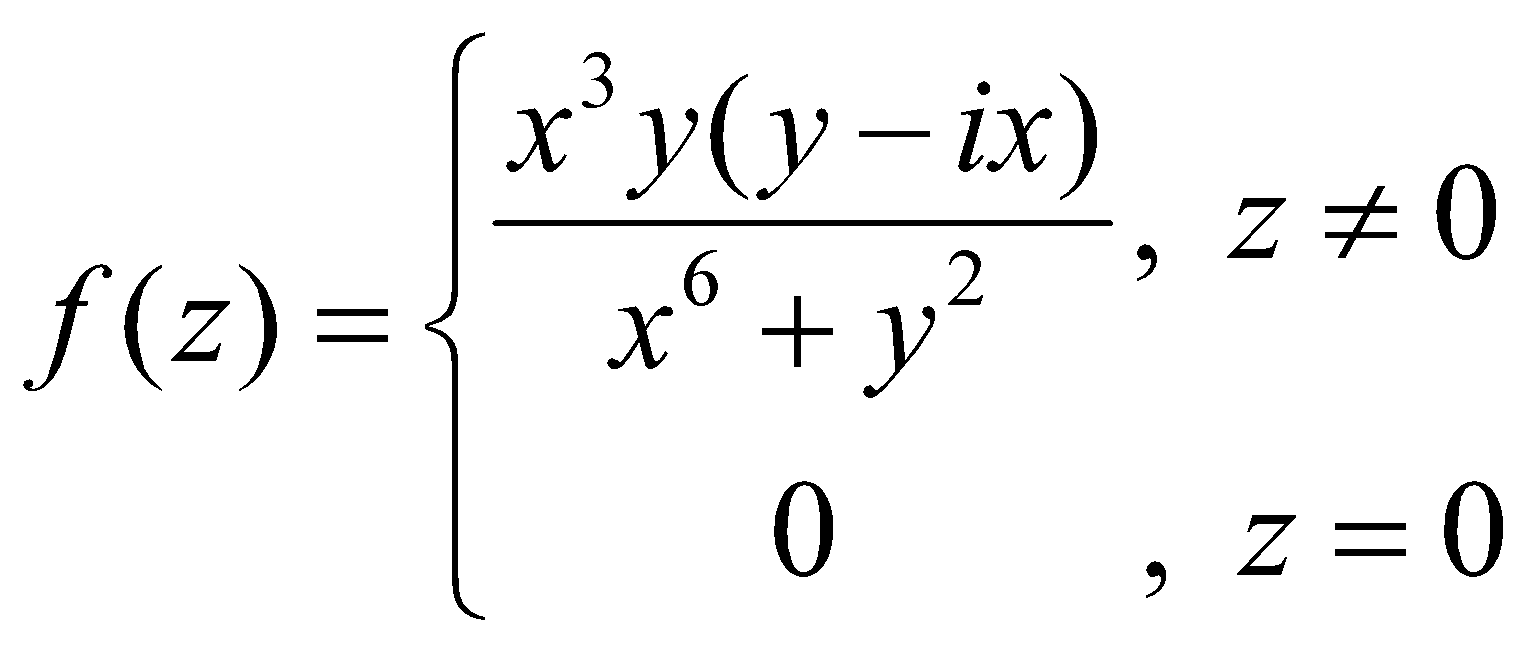Then prove that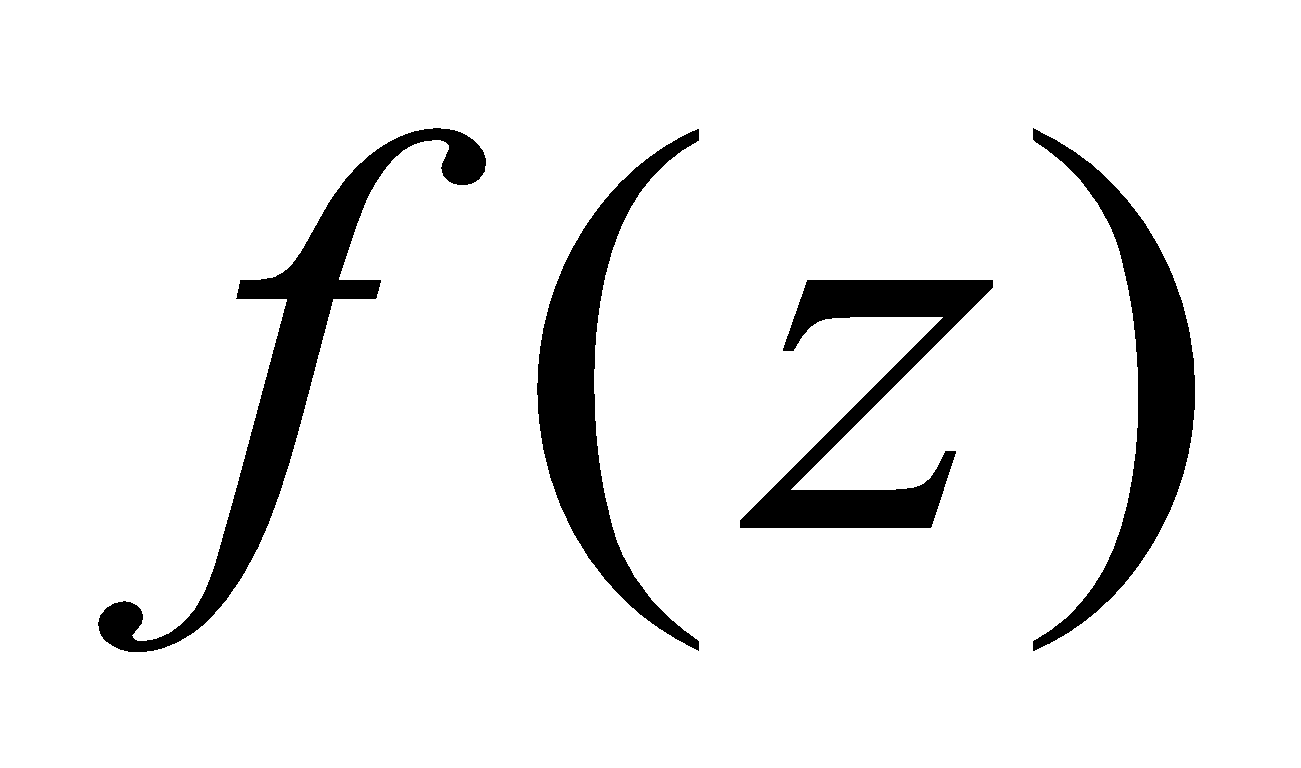is not differentiable at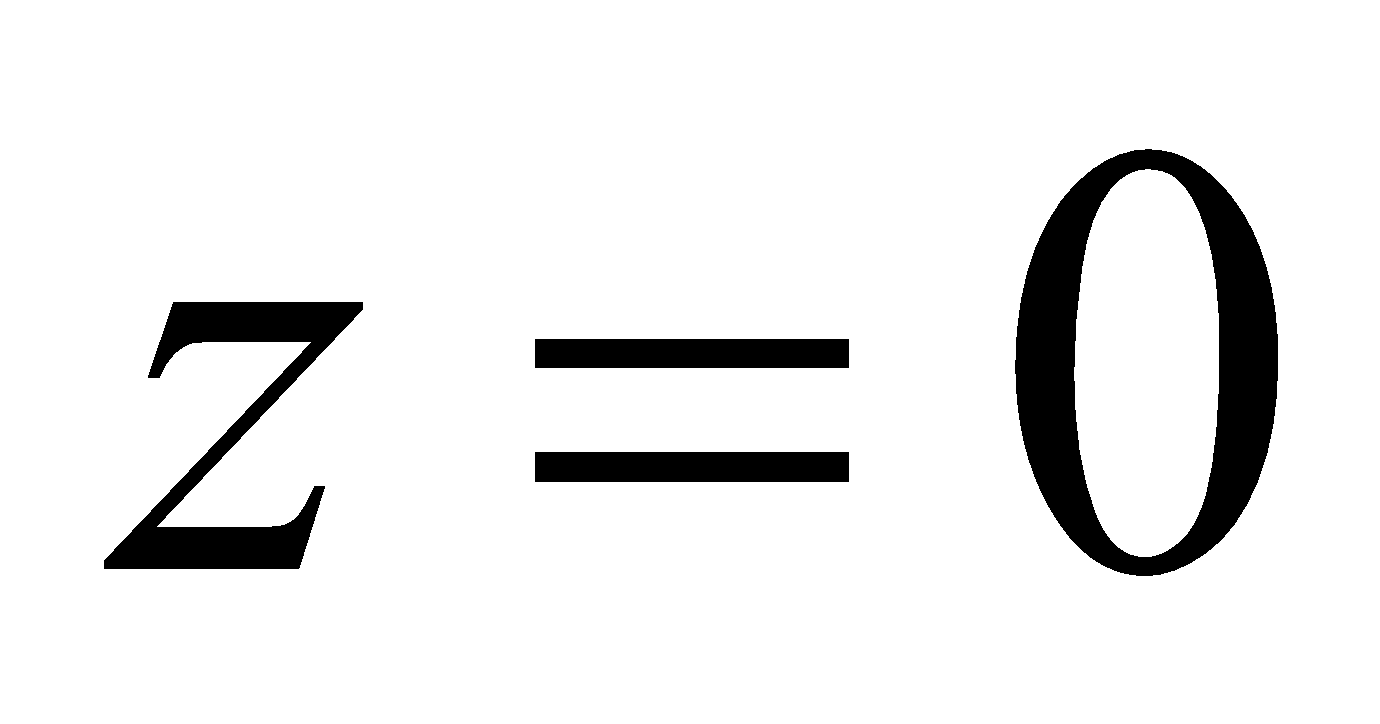. 3
(c) Prove that the function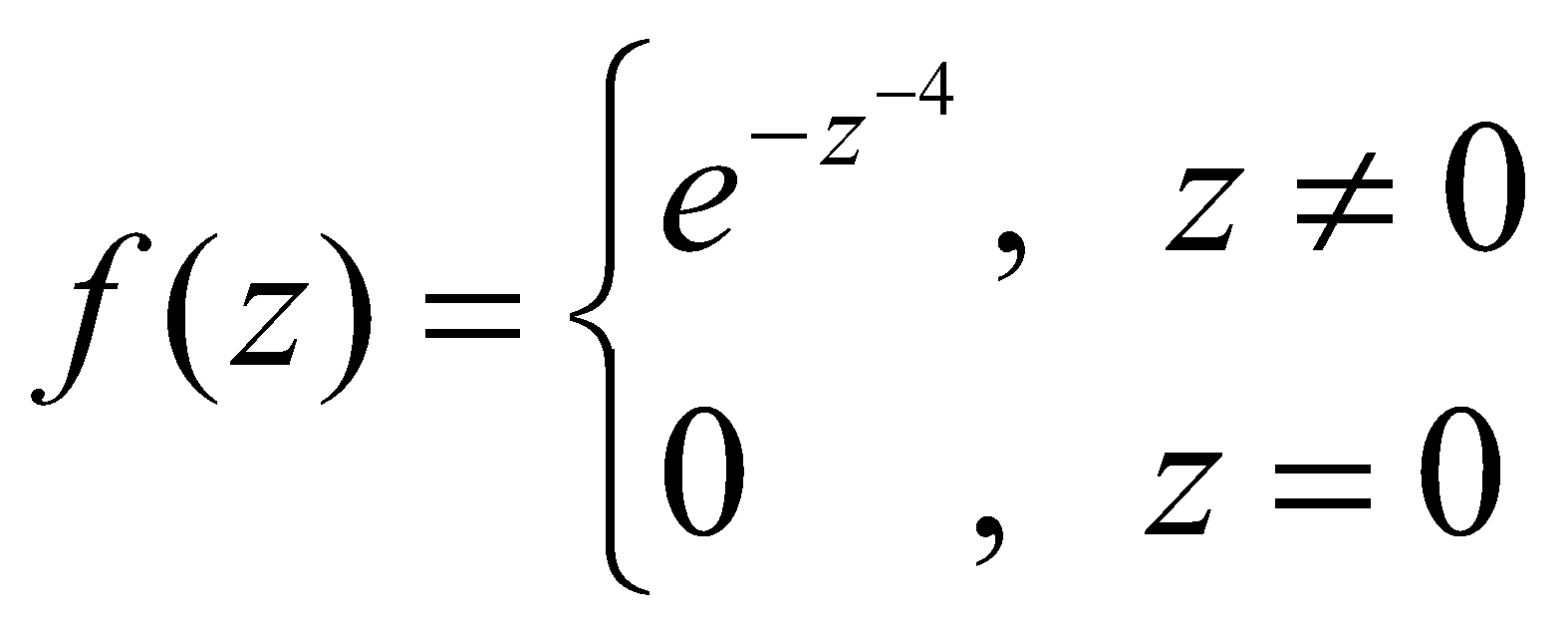is not analytic at, although Cauchy-Riemann equations are satisfied at that point. 6
Or
Prove that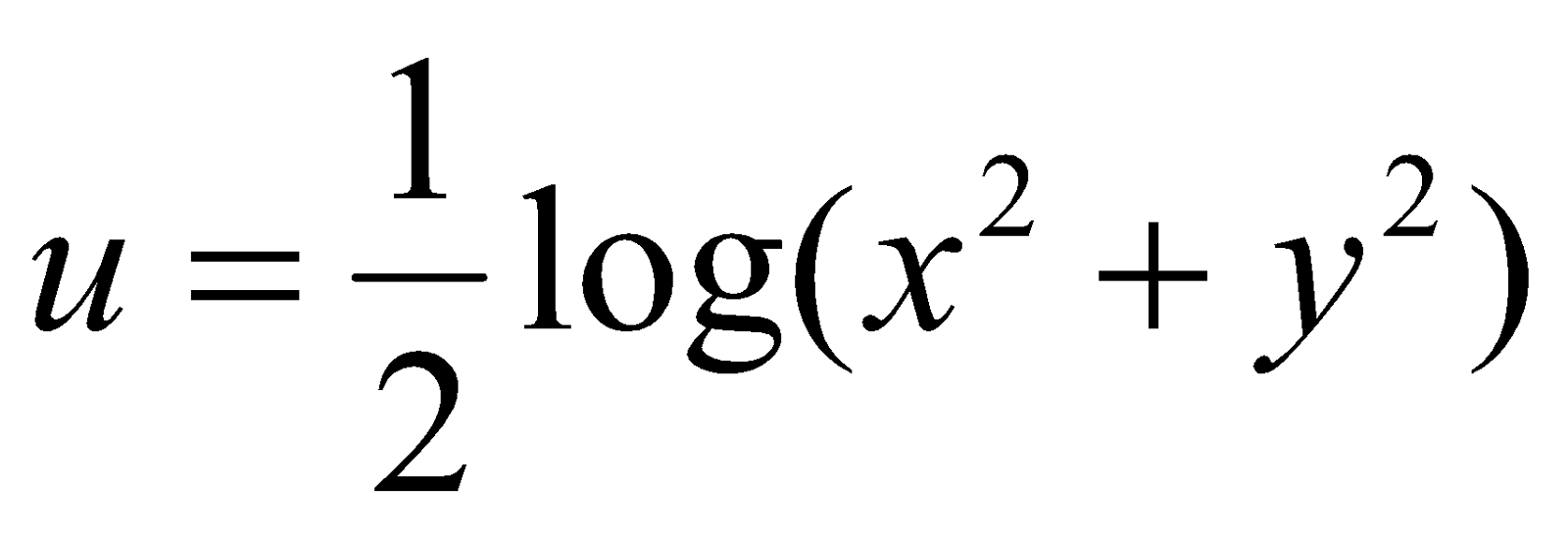is harmonic. Find its harmonic conjugate. 6
6. (a) Define Jordan arc. 1
(b) Evaluate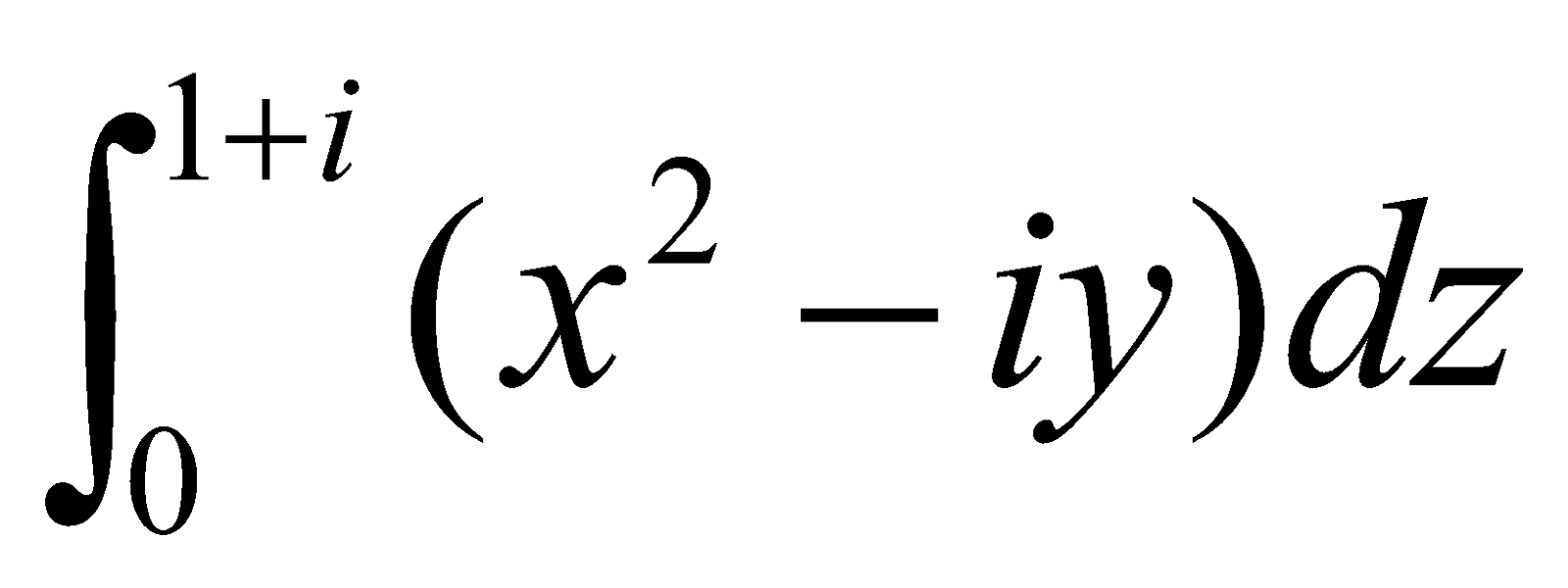along the path 1
1.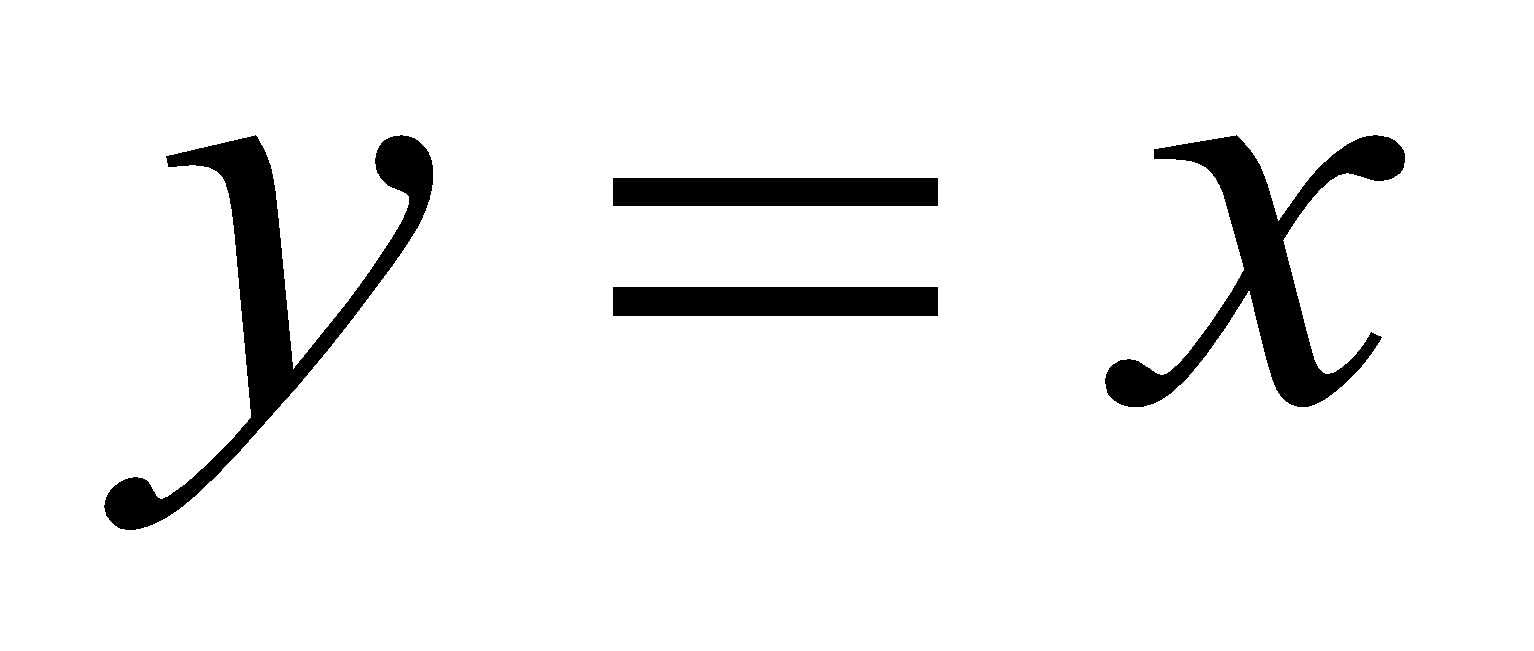2.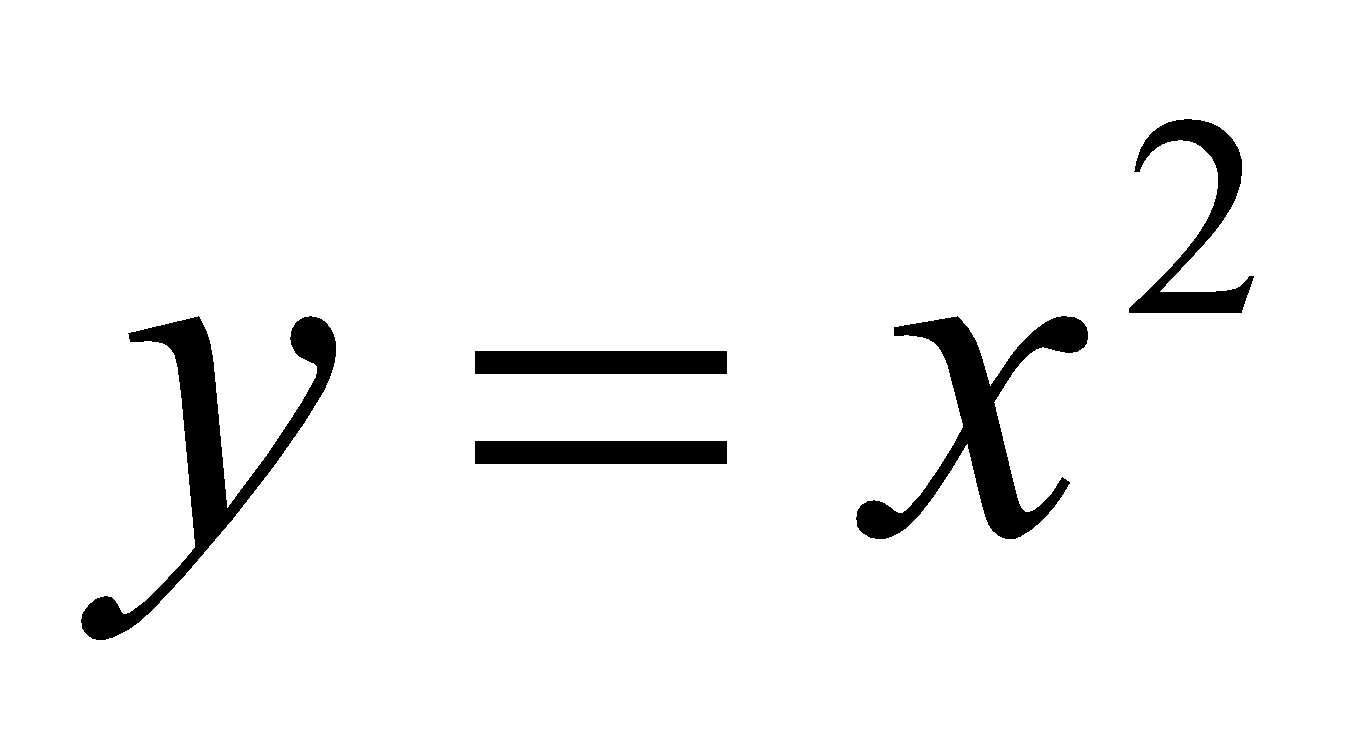(c) State and prove Liouville’s theorem. 5
(d) Answer the following (any one): 4
1. Using Cauchy integral formula, evaluate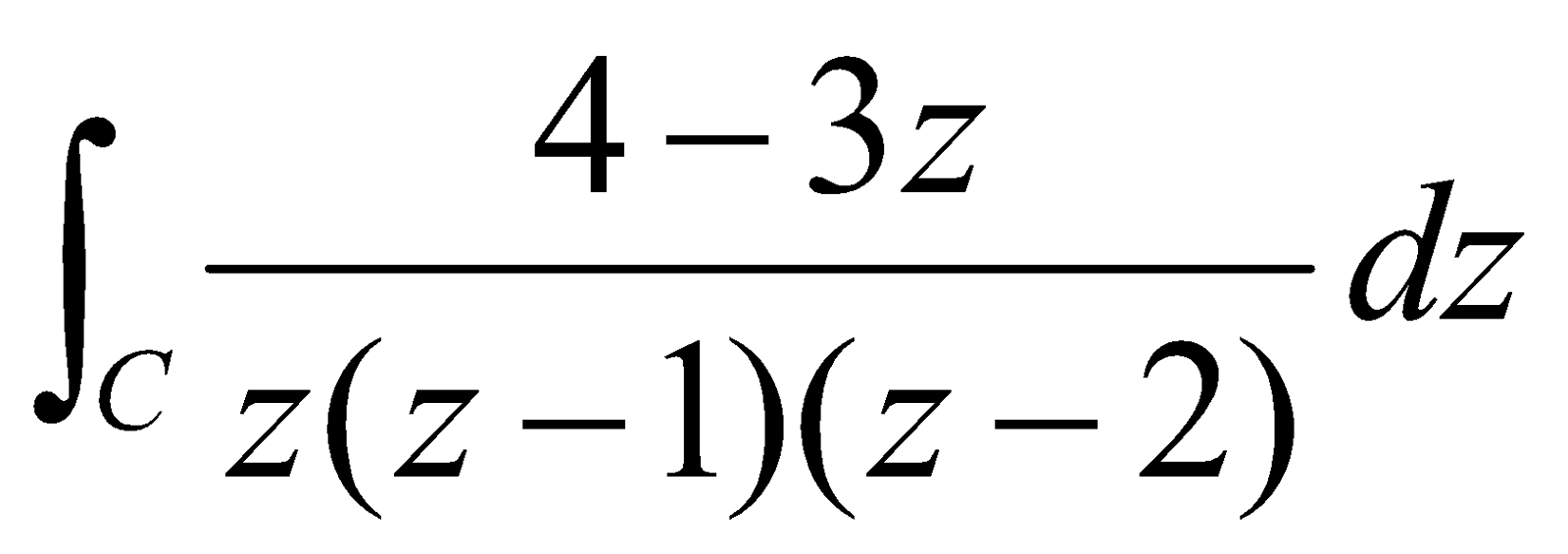where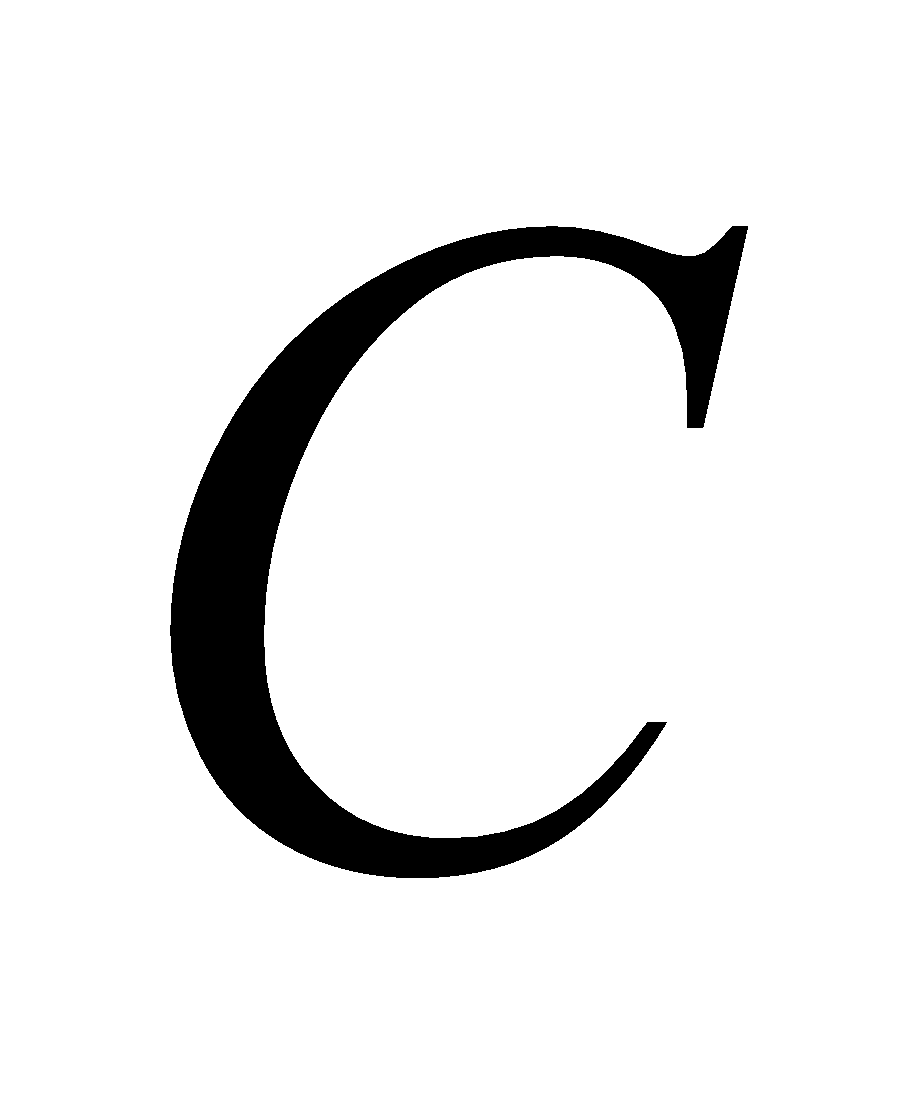is the circle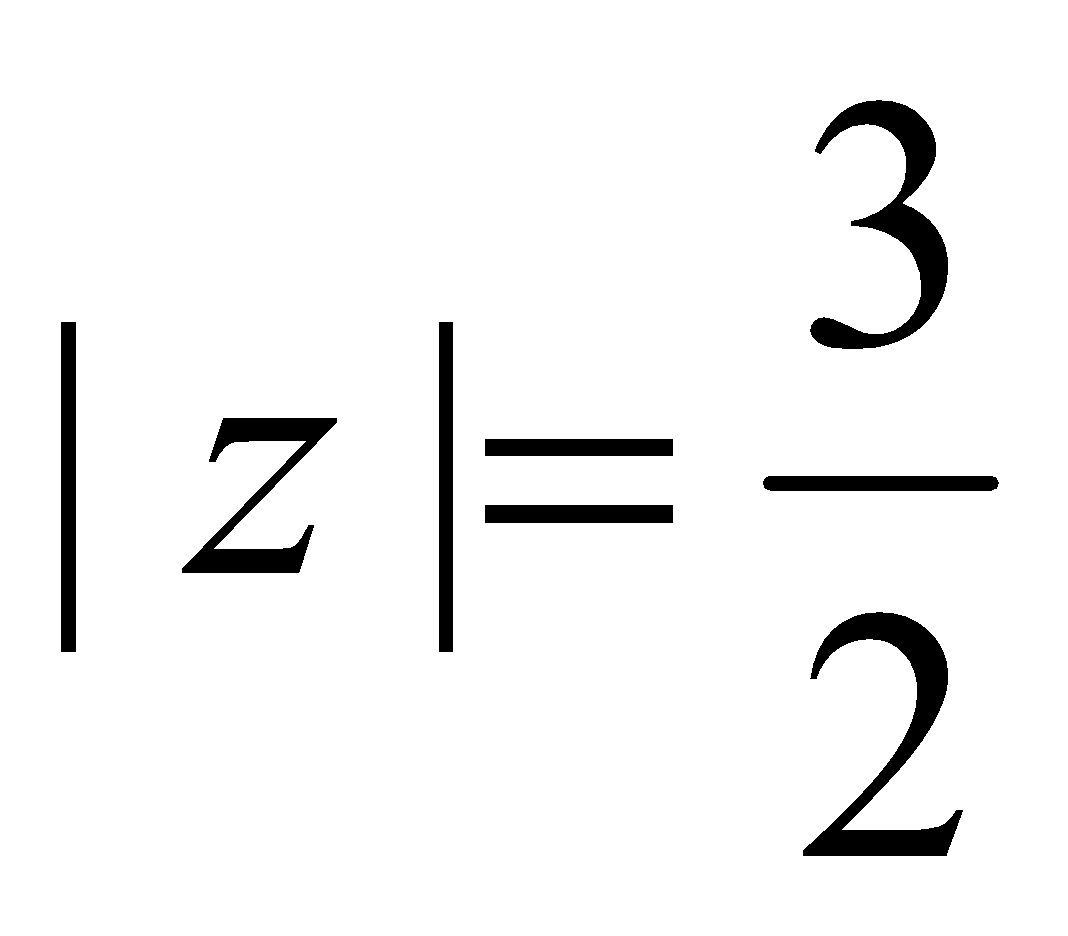.
1. Evaluate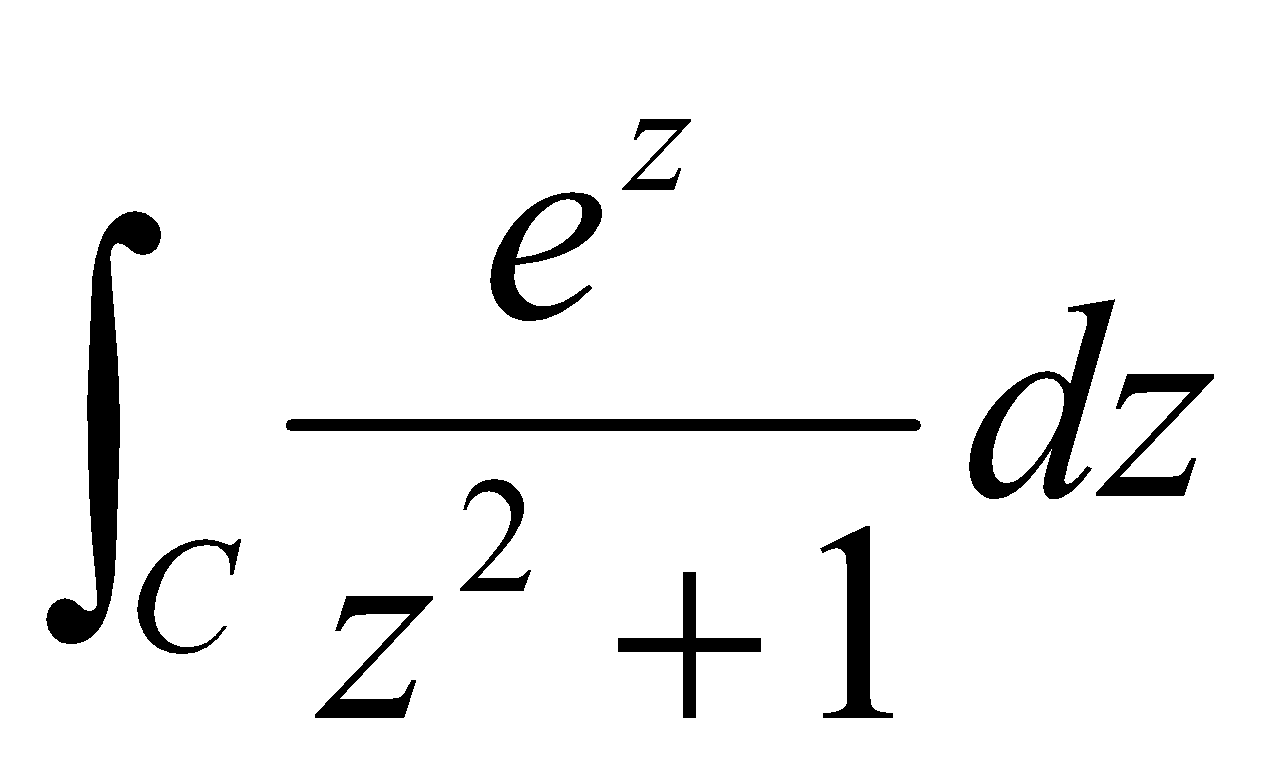, whereis given by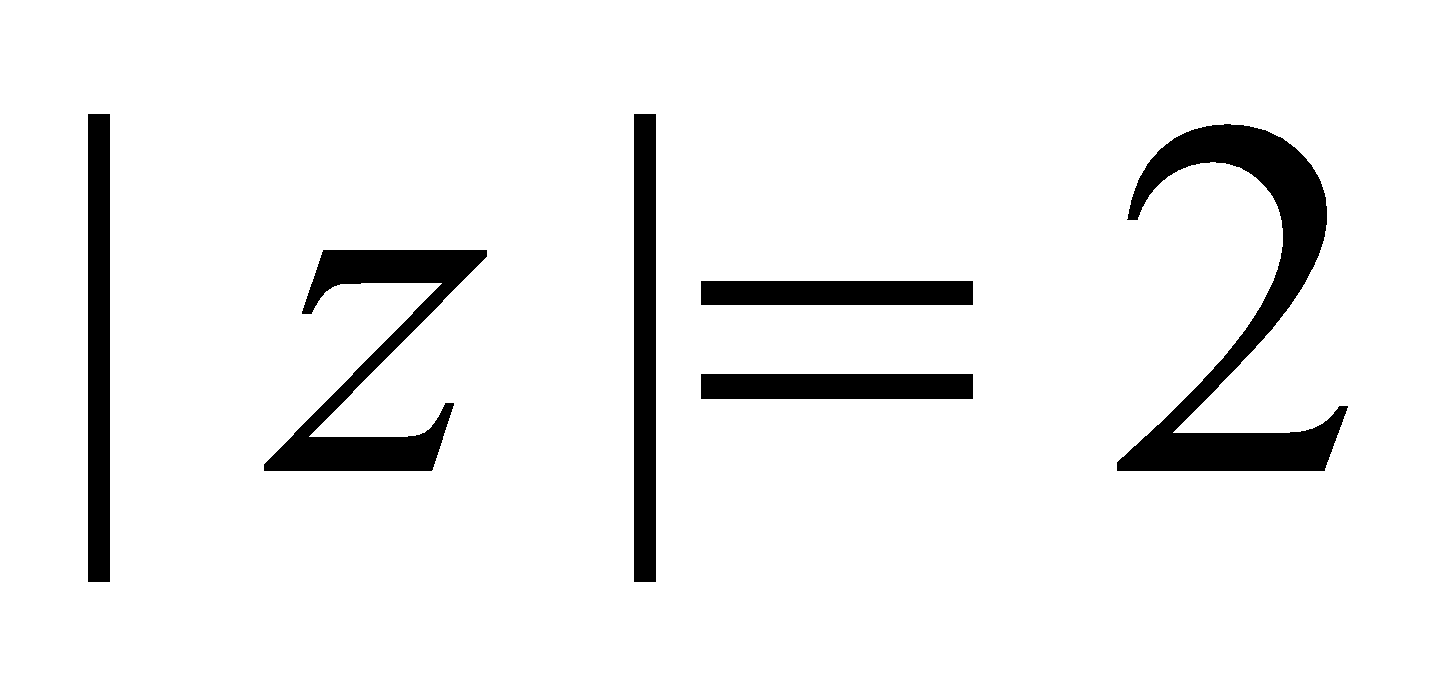.
7. (a) Define radius of convergence of a power series. 2
(b) Expand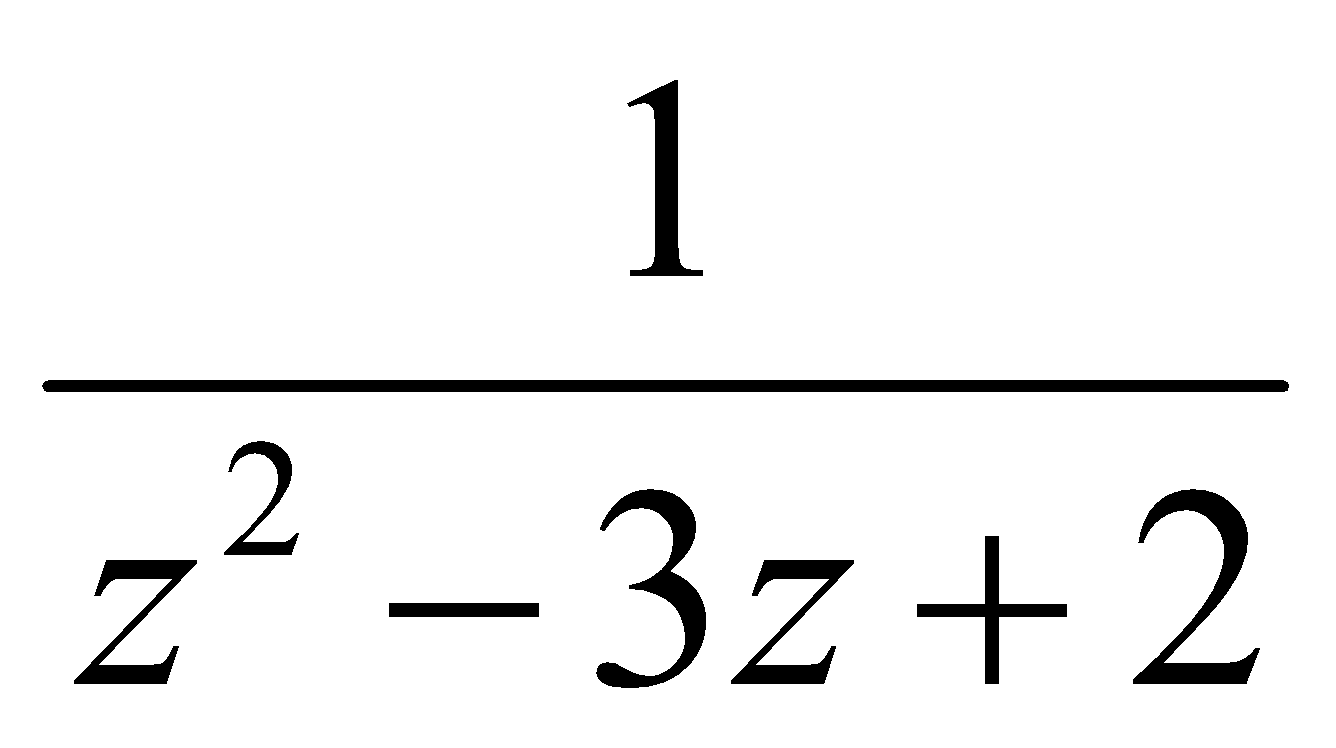in the region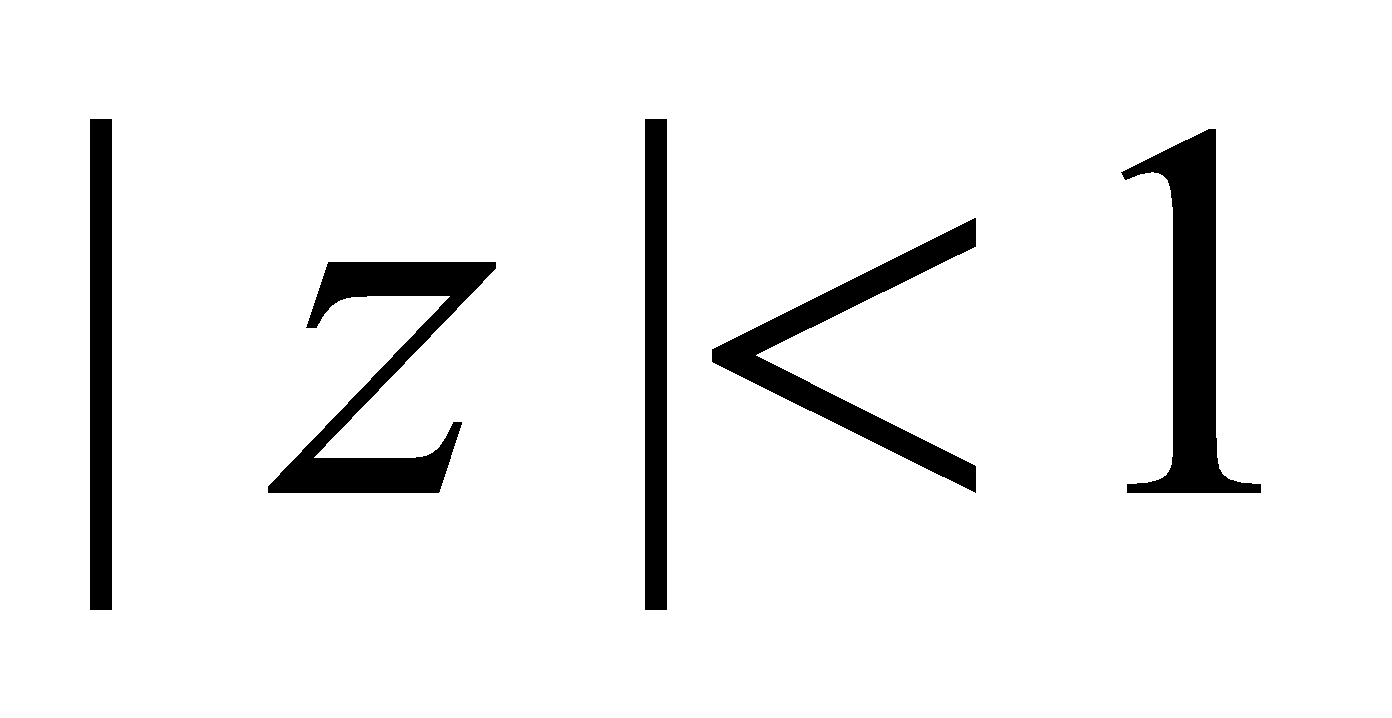. 3
(c) Expand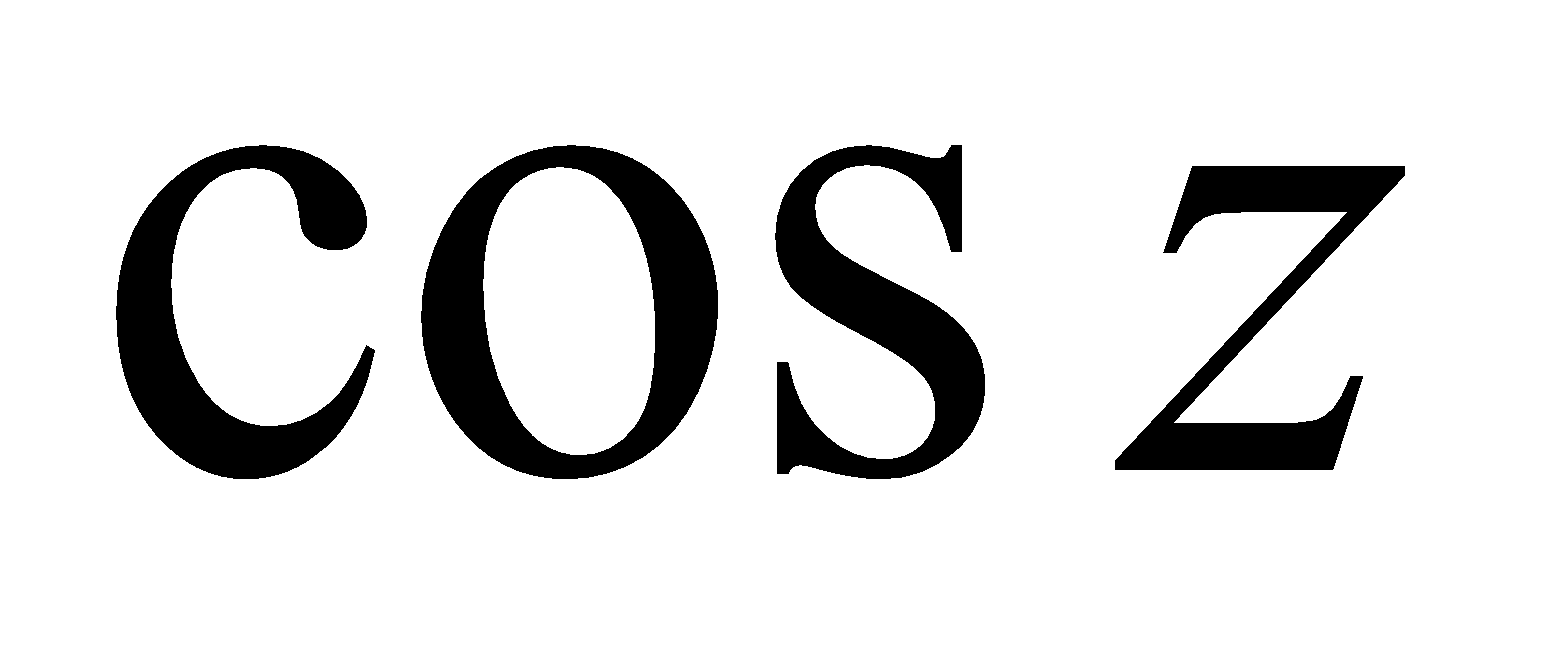in a Taylor’s series about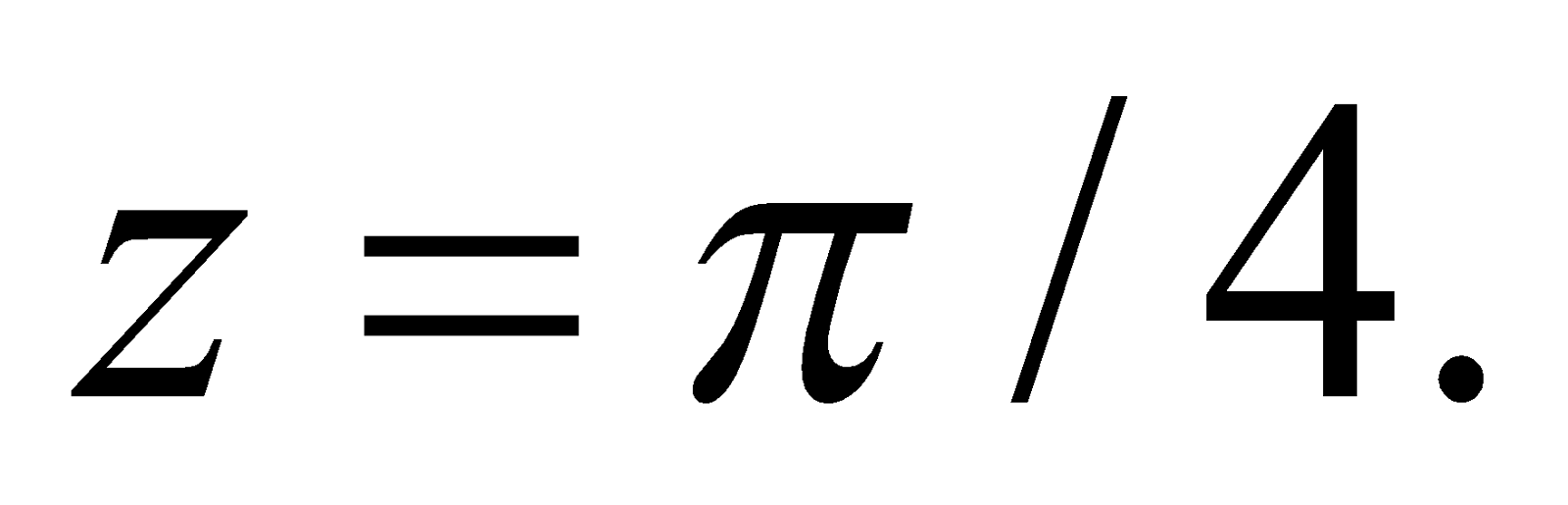3
Or
Expand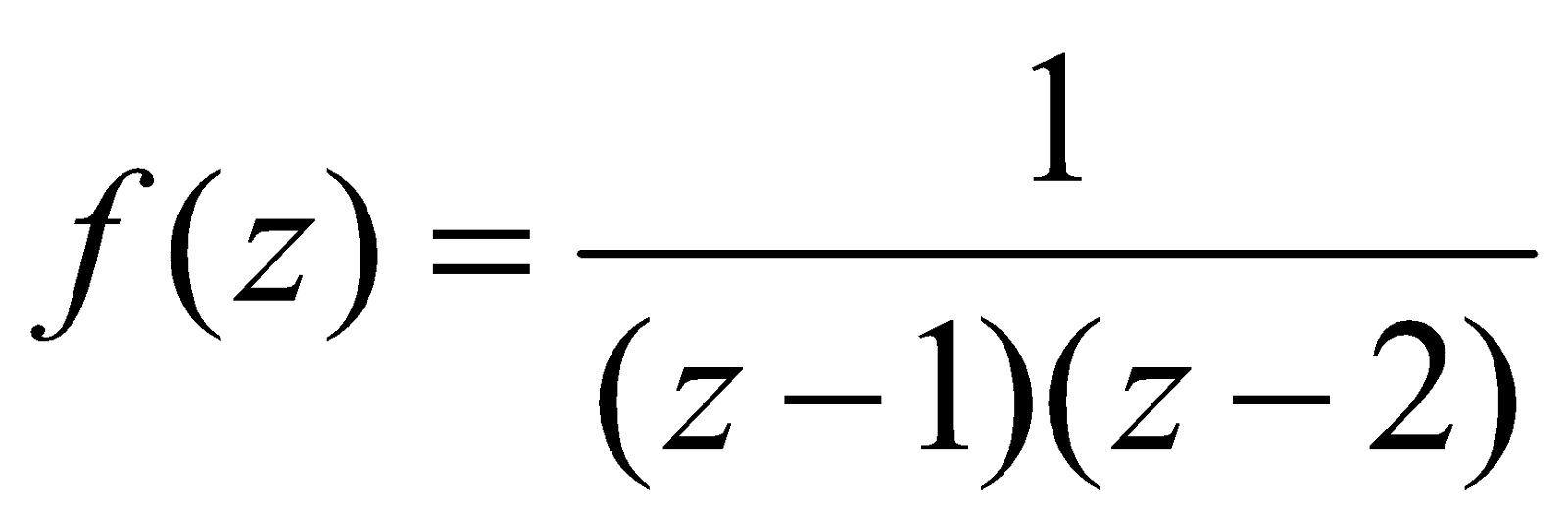for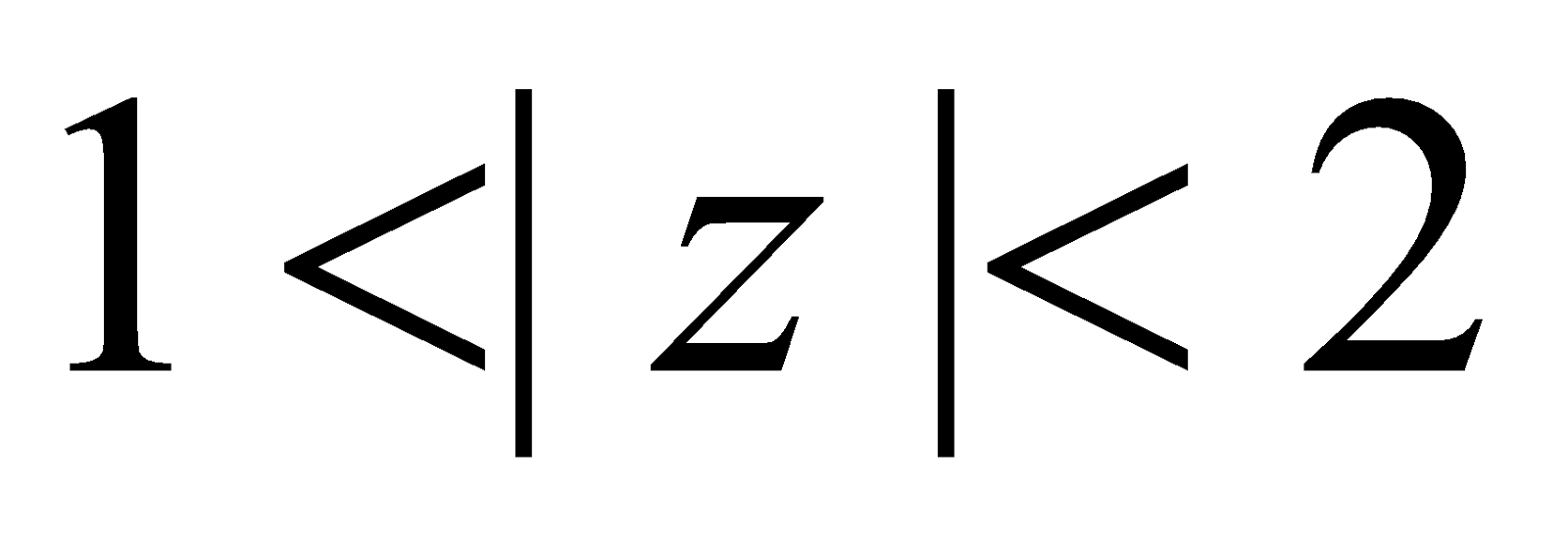. 3
8. (a) Define isolated singular point. 1
(b) Find the poles of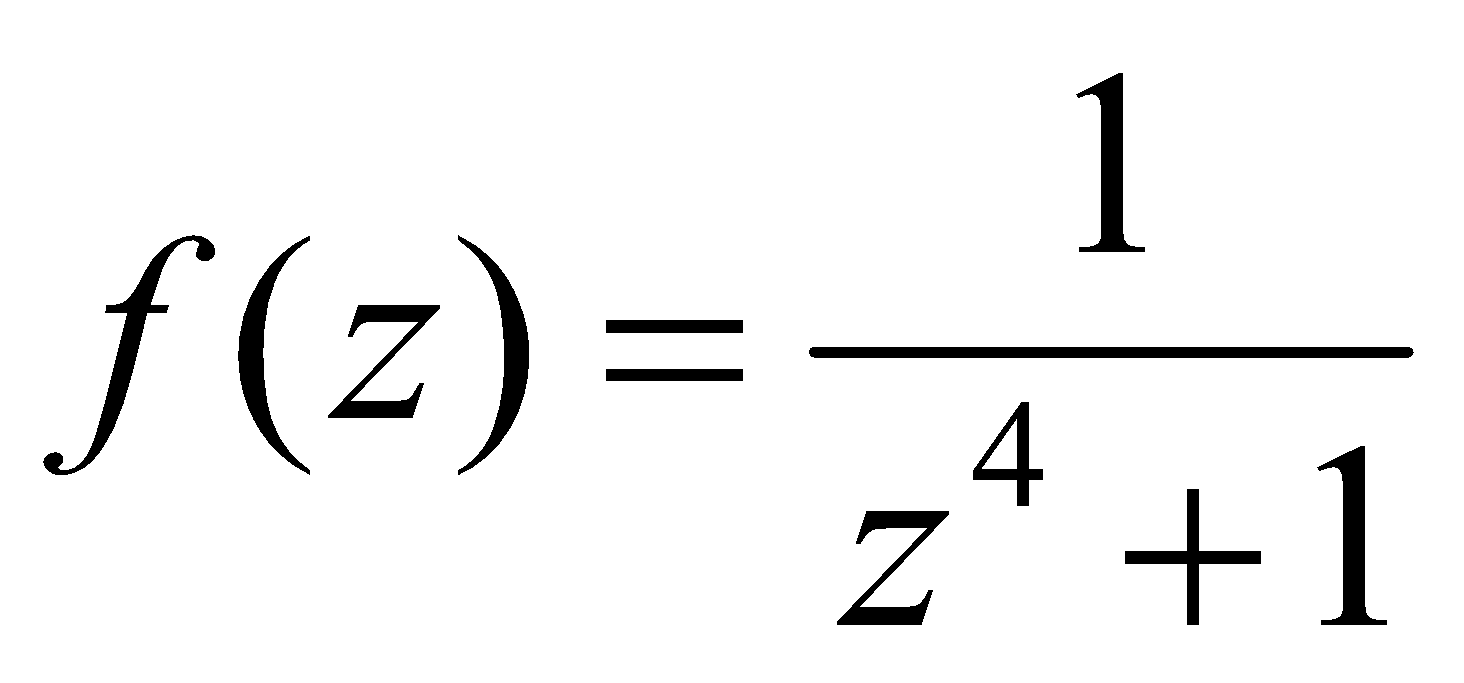. 2
(c) Evaluate (any two): 5x2=10
1.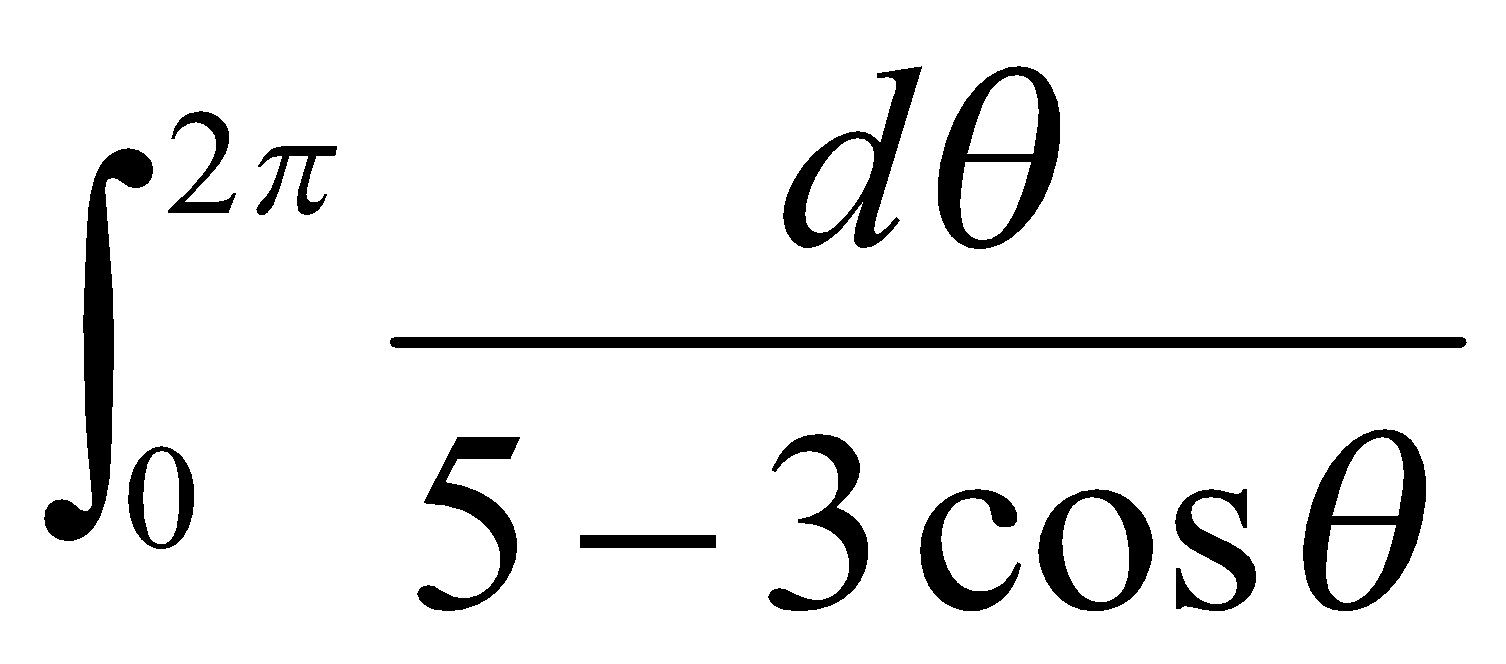2.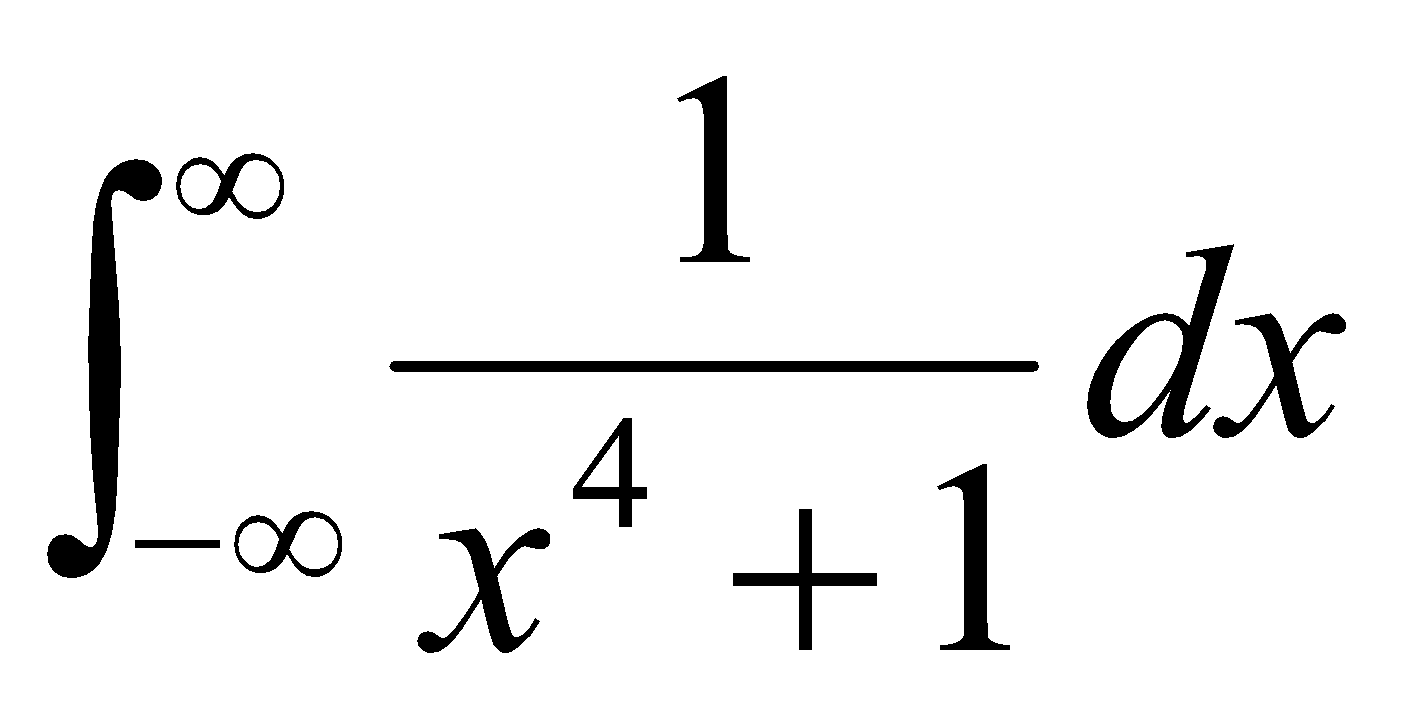3.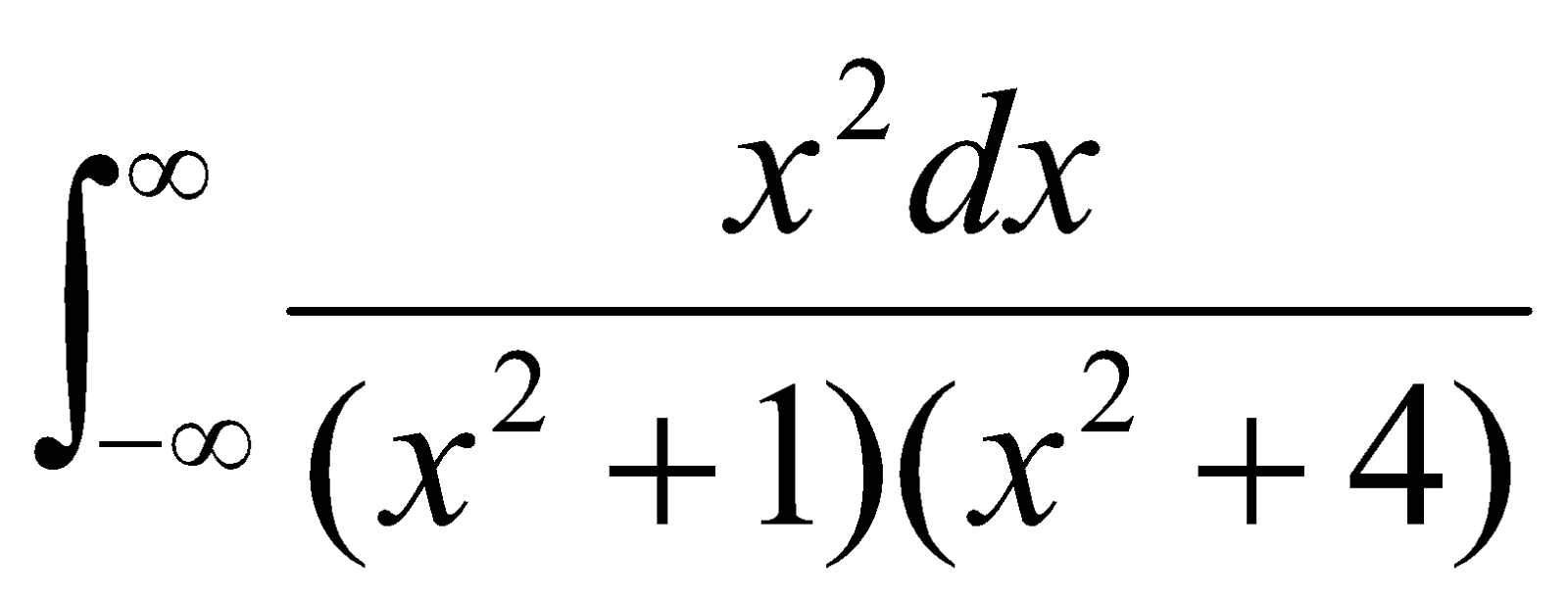4.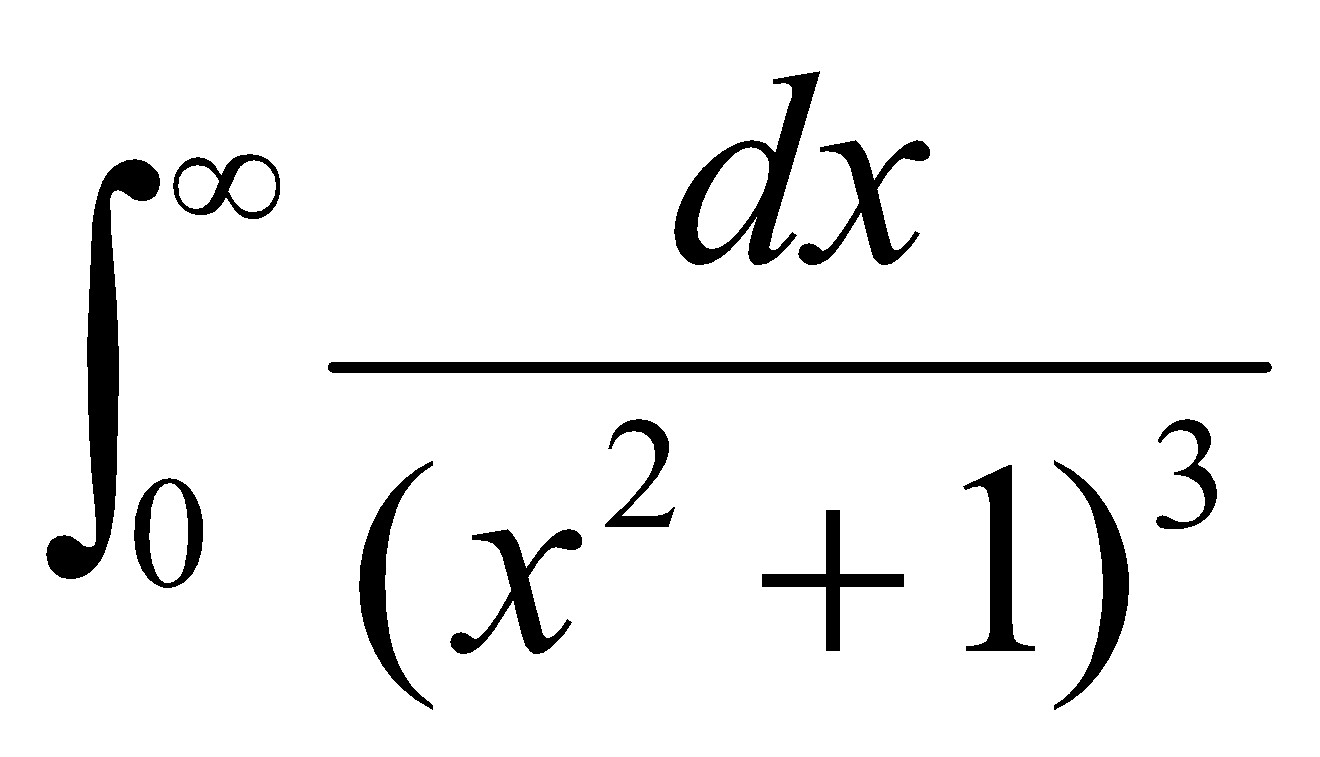***